Jekyll2022-09-30T06:45:11+00:00https://bayes.net/feed.xmlbayes.netTom Adamczewski's blogThe relative importance of hardware and software progress: evidence from computer chess2022-09-21T00:00:00+00:002022-09-21T00:00:00+00:00https://bayes.net/computerchess

I did this analysis in September 2018. Soon after, my career took a turn away from this topic, and I never bothered to publish it. In September 2022, I finally got around to sharing it here. This page is simply the unmodified LaTeX file I used at the time, converted to Markdown using Pandoc. Some tables are included as screenshots because it was simpler that way.

I don't want to invest the time to vet this piece; it's plausible that it contains a mistake, or is otherwise embarrassing. It's a snapshot from 2018.

Given the increase in attention to AI forecasting in recent years, I also suspect this piece is well behind the state of the art today.

# Summary

One topic within AI forecasting is: what is the relative importance of hardware and software to AI progress (Grace 2015, 2017)?

In order to make such forecasts, one option is to look at past events in a relevant reference class. In this document, I present new evidence on the relative importance of hardware and software in explaining the last 33 years of progress in computer chess. I construct and analyse a novel dataset using previously unexploited raw data of 54,919 games organised by the Swedish Computer Chess Association between 1985 and 2018.

This dataset contains instances of the same chess programme run on a range of hardware setups, as well as cases where a single piece of hardware was used to run many different programmes. This allows me to isolate the independent effect of hardware and software on performance.

One approach is to use dummy variables to directly capture the effect of each chess programme, controlling for clock speed. This approach estimates the effect of a clock speed doubling at 76 Elo. As for the dummy coefficients, they tell us, for example, that the software improvement from Fritz 1 to Deep Fritz 8 was 462 Elo. Further interpreting these numbers would require enough background knowledge of computer chess to develop an intuitive sense of how much intellectual progress particular algorithms represented.

A second approach introduces the date a programme was released as a variable, instead of dummies. Because I measure only a proxy for the date of release, this estimate is noisier, but it is more readily interpretable. The date-based estimate suggests that every additional year of chess programme development produces a gain of 10 to 20 Elo, while every clock speed doubling produces an increase of between 69 and 120 Elo, depending on the specification. In a CPU database covering 1971-2014, a clock speed doubling occurs every 3.46 years, suggesting that hardware has been roughly twice as important as software in explaining historical chess progress.

This echoes previous findings that ‘gains from algorithmic progress have been roughly fifty to one hundred percent as large as those from hardware progress’ (Grace 2013). However, the present report goes beyond previous analyses in two ways. First, it ensures that Elo score comparisons are meaningful by using data from a single chess rating list. In addition, it uses multiple regression to more systematically analyse a larger dataset covering a longer time period.

# The Elo system

Performance in chess is traditionally measured using Elo scores. In the Elo system, according to Wikipedia,

Performance isn’t measured absolutely; it is inferred from wins, losses, and draws against other players. Players’ ratings depend on the ratings of their opponents, and the results scored against them. The difference in rating between two players determines an estimate for the expected score between them. […] A player’s expected score is their probability of winning plus half their probability of drawing.

If Player A has a rating of $$R_A$$ and player B a rating of $$R_B$$, the expected score of Player A is $$E_A=(1 + 10^{(R_B-R_A )/400})^{-1}$$.

When a player’s actual tournament scores exceed their expected scores, the Elo system takes this as evidence that player’s rating is too low, and needs to be adjusted upward. Similarly when a player’s actual tournament scores fall short of their expected scores, that player’s rating is adjusted downward. Elo’s original suggestion, which is still widely used, was a simple linear adjustment proportional to the amount by which a player overperformed or underperformed their expected score. The maximum possible adjustment per game, called the K-factor, was set at K = 16 for masters and K = 32 for weaker players.

Supposing Player A was expected to score $$E_{A}$$ points but actually scored $$S_{A}$$ points. The formula for updating their rating is $$R_{A}^{\prime }=R_{A}+K(S_{A}-E_{A})$$.

This update can be performed after each game or each tournament, or after any suitable rating period. An example may help clarify. Suppose Player A has a rating of 1613, and plays in a five-round tournament. He or she loses to a player rated 1609, draws with a player rated 1477, defeats a player rated 1388, defeats a player rated 1586, and loses to a player rated 1720. The player’s actual score is (0 + 0.5 + 1 + 1 + 0) = 2.5. The expected score, calculated according to the formula above, was (0.51 + 0.69 + 0.79 + 0.54 + 0.35) = 2.88. Therefore, the player’s new rating is (1613 + 32(2.5 - 2.88)) = 1601, assuming that a K-factor of 32 is used. Equivalently, each game the player can be said to have put an ante of K times their score for the game into a pot, the opposing player also puts K times their score into the pot, and the winner collects the full pot of value K; in the event of a draw the players split the pot and receive K/2 points each.

# Simpler approaches and why they fail

## Approaches which use only variation in software cannot compare the effects of software and hardware

CCRL (Computer Chess Rating Lists) is an organisation that tests computer chess programmes’ strength by playing the programmes against each other. Each programme is given the same thinking time, or “time control”. On the CCRL 40/40 list, the programmes have the equivalent of 40 minutes for 40 moves on a AMD X2 4600+ processor at 2.4GHz. The programme Crafty 19.17 BH is run as a benchmark on the tester’s computer to determine the equivalent time control for their machine.

All programmes on the CCRL list use equivalent hardware, so any difference in their performance can be attributed to software. Grace (2013) writes:

To confirm that substantial software progress does occur, we can look at the CCRL (2013) comparison of Rybka engines. Rybka 1.1 64-bit was the best of its time (the year 2006), but on equivalent hardware to Rybka 4.1 it is rated 204 points worse (2,892 vs. 3,102)

So we can estimate the increase in Elo scores on the CCRL 40/40 list that is due to improvements in software. But we still don’t know how this compares to the improvements that have come from hardware. For this we need variation in both software and hardware. Furthermore, we need variation in both hardware and software within a single chess ratings list. It may not be valid to compare the Elo improvement from software in one list to the Elo improvement from hardware estimated from a different list1. This is because Elo scores cannot be directly compared across lists. The Elo system only measures the relative performance of players. In human FIDE (World Chess Federation) chess, Elo ratings are able to be calibrated on a single scale, because the same humans play under identical conditions across different tournaments (there is a single time control for all major FIDE events). According to Wikipedia, computer chess rating lists have no direct relation to FIDE Elo ratings:

there is no calibration between any of these [computer] rating lists and [human] player pools. Hence, the results which matter are the ranks and the differences between the ratings, and not the absolute values. Also, each list calibrates their Elo via a different method. Therefore, no Elo comparisons can be made between the lists.

For example, in the CCRL 40/4 list, the top rated programme has an Elo of 3560, while the current champion of the 40/40 list, which allows 10 times more thinking time, has an Elo of only 3439.

## Estimating software progress as what is left unexplained by hardware suffers from omitted variable bias

Supposing we had data from a single ratings list with variation in both software and hardware, we might reason as follows. A chess programme is a piece of software run on some hardware, so hardware and software are jointly exhaustive categories of inputs to chess performance. Hence whatever difference in performance isn’t explained by hardware must be due to software, and vice versa. One could run a regression of Elo scores on some measure(s) of hardware (say, processor clock speed and RAM), the residuals of which would be a hardware-adjusted Elo score. Any change in hardware-adjusted Elo scores would be due to software. However, this line of reasoning is flawed. Hardware is not exogenously determined, it is correlated with the residual “software” term in the regression. Modern chess programmes are run on much better hardware, So the regression would suffer from omitted variable bias in the direction of overestimating the effect of hardware.

# This document’s approach

## The SSDF dataset

On computer chess ranking lists, hardware is usually scrupulously standardised in order to compare chess programmes in the fairest way possible.

I could find only one source of data in which hardware varies: the SSDF (Swedish Computer Chess Association) list, which records chess games since 1985 and has changed its hardware several times. I process and merge SSDF’s raw data to produce a novel dataset of 366 programmes-hardware pairs and their Elo ratings. The data methods are detailed in section 5.

## Measuring software

If we want to include software as an independent variable, we need to measure the “quality of software” of each chess programme in a meaningful and interpretable way. I use two different approaches.

### Using programme dummies

When SSDF changed their hardware in 2008, they commented2:

Six [programmes] have been tested on both Q6600 and Athlon 1200 MHz, which makes a comparison possible. The total effect of a faster processor, four instead of one CPU and the use of 64-bit operating system instead of 32 bit has in average given a rating increase of 120 points. Deep Fritz 8 gained the most with 142 points whereas Deep Junior 8 increased the least with 84 points.

This example allows us to compare the gains due to hardware (Athlon to Q6600) to the gains due to software (e.g. Deep Fritz 8 to Deep Rybka 3). We have a 2x2 table of Elo scores:

Q6600 Athlon
Deep Rybka 3 3193 3075
Deep Fritz 8 2898 2781

The generalisation of this approach is to conduct a regression in which $$n-1$$ dummy variables are created for $$n$$ programmes in the dataset. This allows us to quantify the effect of moving from some “baseline programme” (which has no dummy) to any other programme, controlling for hardware.

I am able to identify 46 instances where a programme was used on more than one hardware configuration, totalling 96 data points (because four programmes were used on three configurations). Out of these, 82 have clock speed data available3. I use the natural log of clock speed throughout, in keeping with Moore’s law. I use Fritz 1 as the baseline programme.

Table 1 presents the results. As expected, this regression explains virtually all the variation, since hardware and software are jointly exhaustive categories of computer chess inputs. The regression coefficient on clock speed is estimated with very high precision.

Since we are using log clock speed, the interpretation of the coefficient is that for every clock speed doubling, $$\ln(2)*110 \approx 76$$. This is about the same as the difference between Conchess Glasgow and Fritz 1. For comparison, data from the Stanford CPU Database (Danowitz et al. 2012), which covers 1971-2014, suggests that a clock speed doubling has historically occurred every 3.46 years.

We can see the progression of the Fritz engines, controlling for clock speed:

Improvement from Fritz 1 Release Date
Fritz 1 0 1992
Fritz 3 192 1995
Deep Fritz 395 2000
Deep Fritz 7 428 2002
Deep Fritz 8 462 2004

The Fritz line of software has improved by about 39 Elo per year on average, suggesting that software has been responsible for almost twice as much progress as hardware when it comes to Fritz.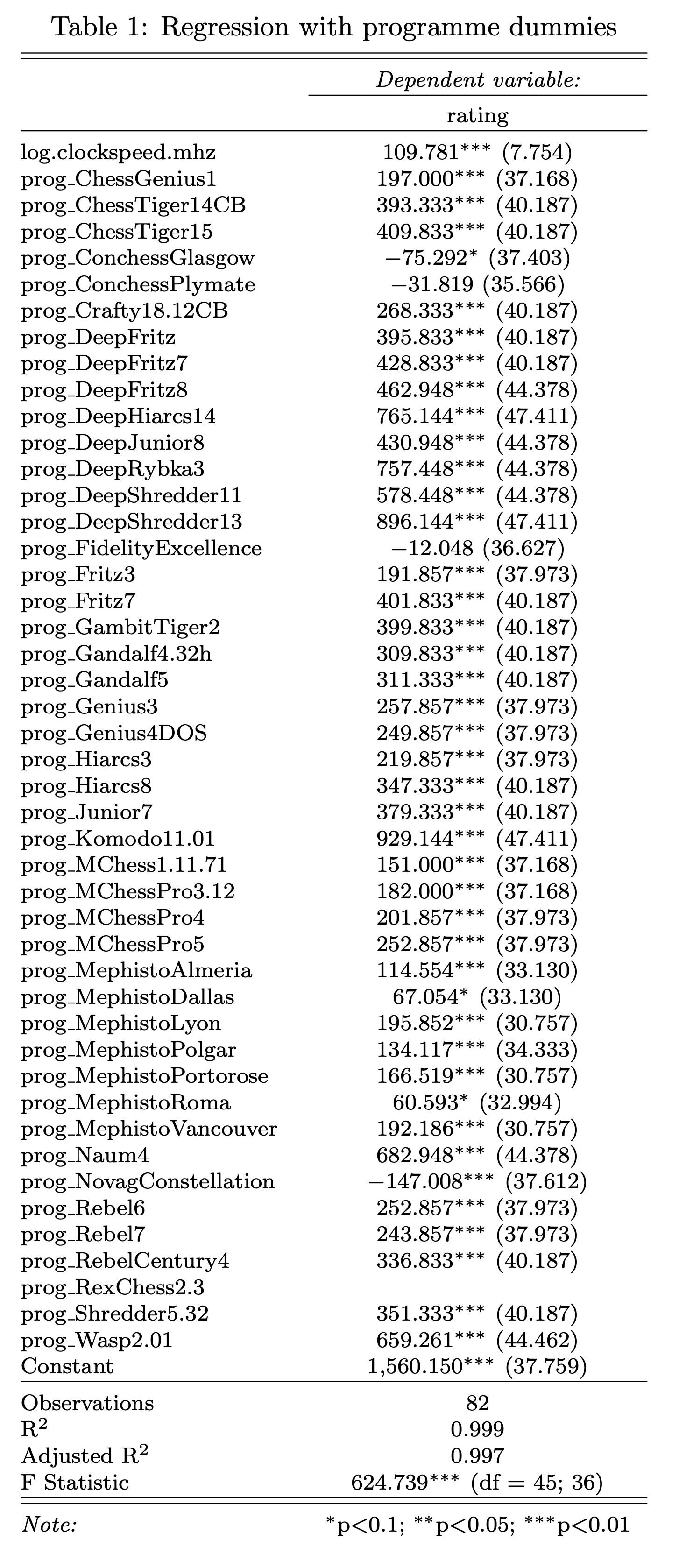### Using the date of release

Another approach is to use the release date of a programme as a (very noisy) measure of the amount of developer effort that went into its design. There are two main advantages to this approach. First, more data is available since we are not restricted to instances where a programme was used on more than one hardware configuration. Second, the regression coefficient is easily interpretable as measuring the effect of one year of computer chess development on performance. The main disadvantage is the noisiness of the proxy.

To approximate the release date of a programme, I use the date at which the programme was first played in an SSDF game. The approximation is very good for the most part, except in some cases where the organisers intentionally test very old “legacy” programmes on new hardware for the first time.

I conduct two regressions, presented in Table 2. Regression (1) ($$n=233$$) uses release date and clock speed only. Regression (2) ($$n=138$$) employs a finer-grained measure of hardware that includes clock speed, RAM, and the product of clock speed and number of cores (total speed). All coefficients are estimated with very high statistical precision.

It appears that after accounting for clock speed, the number of cores tells us little about performance, while the coefficient on RAM is actually small and negative. This could be because the small amount variation in RAM and the number of cores in this dataset (see section 5.2.3) is highly correlated with changed in clock speed. The effect of clock speed is of similar magnitude to that found using dummies: between 69 and 120 Elo per doubling. The effect of the release date is between 10 and 20 Elo points per year. Recall that a clock speed doubling has historically occurred every 3.46 years (Danowitz et al. 2012). This would suggest that hardware has mattered roughly twice as much as software for progress in computer chess.# Data processing methods

## Sourcing and importing the data

### SSDF ranking

The file ssdf-summary-data-original.txt contains the current rating of 366 programme-hardware pairs in tab-separated plain text format, as well as information on hundreds of games. I truncate the file to retain only the ranked list, and convert it to CSV ssdf-summary-data-clean.csv. I call this dataset su.

### SSDF data on 54,919 games

I download the plain-text database SSDF.PGN, which fully describes each of the 54,919 games (including every move made by each player). An example entry:

[Event "Testspel av Tony Hed"]

[Site "?"]

[Date "1992.01.01"]

[Round "?"]

[White "Fritz 1 486/33 MHz"]

[Black "Mephisto Academy 6502 5 MHz"]

[Result "0-1"]

[ECO "A28"]

[PlyCount "83"]

1. c4 e5 2. Nc3 Nf6 3. Nf3 Nc6 4. d4 e4 5. Ng5 h6 6. Ngxe4 Nxe4 7. Nxe4 Qh4 8. Nc3 Qxd4 9. e3 Qxd1+ 10. Kxd1 Be7 11. Nd5 Bd8 12. Be2 O-O 13. Bd2 d6 14. Bc3 Ne5 15. Ba5 c6 16. Bxd8 Rxd8 17. Ne7+ Kh7 18. Nxc8 Raxc8 19. Ke1 d5 20. b3 dxc4 21. Bxc4 Rc7 22. Rd1 Rxd1+ 23. Kxd1 Rd7+ 24. Kc2 Nxc4 25. bxc4 Kg6 26. Rd1 Rxd1 27. Kxd1 Kf5 28. Ke2 c5 29. h3 a6 30. Kd3 b5 31. f4 g5 32. g3 bxc4+ 33. Kxc4 g4 34. e4+ Kxe4 35. hxg4 f6 36. Kxc5 Kf3 37. g5 hxg5 38. Kd5 Kxg3 39. fxg5 fxg5 40. Kc5 g4 41. Kb6 Kf4 42. Kxa6 0-1

I write the python script extract-earliest-dates.py, which uses regular expressions to clean up the file. In the resulting output3.txt, there is one line for every game; each lines gives the date and the programme-hardware pair which played White, separated by a comma. I call this data full.

## Data analysis in R

### Cleaning both data sets

All subsequent data cleaning and analysis are conducted in r.R. In the source data, the software-hardware pairs are in unstructured plain text. For example:

Rebel Century 3 K6-2 450 MHz

P.Fritz 3 Glaurung 2.1 PXA270 520 MHz

Pocket Fritz 2 Shredder PXA255 400 MHz

Goliath Light K6-2 450 MHz

Fritz 5.32 64MB P200 MHz MMX

Crafty 17.07 CB K6-2 450 MHz

Nimzo 99 K6-2 450 MHz

Resurrection Rybka 2.2 ARM 203 MHz

MChess Pro 8 K6-2 450 MHz

Genius 6.5 K6-2 450 MHz

Chessmaster 6000 64MB P200 MHz MMX

Hiarcs 7.32 64MB P200 MHz MMX

Fritz 5 PB29% 67MB P200 MHz MMX

ChessGenius 3 ZTE Apex3 ARM A53 1.3 GHz

Fritz 4 Pentium 90 MHz

Kallisto 1.98 Pentium 90 MHz

MChess Pro 5 486/50-66 MHz

Rebel 7 486/50-66 MHz

Significant data cleaning is required. I define 5 functions using regular expressions, which are applied in the order below. For consistency, I apply the same functions to both su and full.

• extract_clock_speeds() uses two different methods. First, it matches strings of the form 2.4 GHz where a decimal number is followed by GHz or MHz. Second, it captures anything to the right of a processor (see extract_processors() below), since clock speeds are usually given immediately after the name of the processor.

• clean_clock_speeds() removes extraneous characters and turns strings with GHz and MHz into numeric values in MHz.

• extract_processors() catches anything that matches a manually collected list of 32 processors.

• standardise_progs() removes whitespace and trailing zeroes. Next, and somewhat contentiously, it removes x64 and MP (“multi-processor”). This is because these labels are inconsistently applied between the two datasets, and to my understanding also within SSDF.PGN. Keeping these labels would make merging much more difficult, and the date-based analysis relies crucially on merging datasets. For the dummies analysis, the choice is less defensible, but allows much more data to be used. The hope is that there is not too much difference between the x64 and MP versions and other versions of a single programme. If I did further work with this data, I would look at the impact of these choices on the results.

• clean_progs() removes extraneous characters

### Processing full

full contains missing dates, indicated using question marks. The function unknown_date_default() deals with missing values in the following way:

• An unknown year defaults to 2100, since we are only interested in the earliest appearance of an programme

• An unknown month or day defaults to 01 (the more principled choice would be 31 for a missing day and 12 for a missing month, but this would require a more cumbersome regular expression, and I don’t need that much precision)

Then I drop all observations that are not the earliest appearance of an programme. I also remove games that are claimed to have occurred in 1900.

### Imputing RAM and number of cores

The number of cores in a processor, and the amount of RAM on the computer that was used for a game are usually not given in the source data (only the clock speed is). This data needs to be added manually. On hardware.htm, the following is written:

The hardware of the different hardware levels:

• Intel Pentium 90 MHz - 8-16 MB RAM

• Intel Pentium 200 MHz MMX - 32-64 MB RAM

• AMD K6-2 450 MHz - Single processor, 32 bit OS, 128 MB RAM, 5 piece TableBases on HHD

• AMD Athlon Thunderbird 1200 MHz - Single processor, 32 bit OS, 256 MB RAM, 5p TB on HHD

• Intel Core2Quad Q6600 2400 MHz - Quad Core processor, 64 bit OS, 2 GB RAM, 5p TB on HHD

• AMD Ryzen 7 1800X 3600 MHz - Octa Core processor, 64 bit OS, 16 GB RAM, 6p Syzygy on SSD (or 5p Nalimov on SSD)

I manually write this in processor-info.csv, to be merged later. Further work on this could involve digging up more such information. Sometimes, when a new version of the list is released, SSDF adds a comment on comment.htm. I have extracted all such comment pages from the internet archive, they can be found in the directory datacomments. I have not studied them, but more information on the hardware setups that were historically used could be hidden there.

### Merging

For the date-based analysis, I now finally merge su and full, matching by the programme name. I also merge the imputed information in ramcores.

For the dummies analysis, I only merge ramcores.

When information on the number of cores is available, I compute the clock speed times the number of cores, which is the total number of operations per second available to the processor.

## Further data

I use the Ruby script mayback_machine_downloader (Hartator 2018) to pull all versions of the entire SSDF site stored on the Web Archive. I have not yet done anything with this data.

When analysing the Stanford CPU database (Danowitz et al. 2012), I use only the summary file processor.csv. I simply regress the date on the natural log of clock speed. Then I multiply the coefficient by $$\ln(2)$$ and divide by $$365$$.

Danowitz, Andrew, Kyle Kelley, James Mao, John P. Stevenson, and Mark Horowitz. 2012. “CPU DB: Recording Microprocessor History.” *Commun. ACM* 55 (4): 55–63. <https://doi.org/10.1145/2133806.2133822>.
Grace, Katja. 2013. “Algorithmic Progress in Six Domains,” 60.
———. 2015. “Research Topic: Hardware, Software and AI.” *AI Impacts*. https://aiimpacts.org/research-topic-hardware-software-and-ai/.
———. 2017. “Effect of Marginal Hardware on Artificial General Intelligence.” *AI Impacts*. https://aiimpacts.org/effect-of-marginal-hardware-on-artificial-general-intelligence/.
1. To the extent that Elos from different lists are not comparable, analyses such as that in section 5.1.3 of Grace (2013) would be invalidated.

2. See datacomments/comment_003.htm

3. I do not use the RAM and cores data I have collected (see section 5), since this would reduce the number of data points even more, but such a regression could easily be conducted.

]]>
How to run Cronicle (a cron replacement) in a Docker container2022-05-07T00:00:00+00:002022-05-07T00:00:00+00:00https://bayes.net/cronicleI really don’t like cron jobs and crontab:

• crontab has a horrible syntax (it’s from 1975…)
• logging the output of jobs needs to be specified manually
• viewing logs is inconvenient (even just for checking whether a job ran or not!)
• cron jobs run in a minimal environment that’s inconvenient to modify

Cronicle is a friendlier alternative (“a task scheduler with a web based front-end UI”).

Very important: the default username/password for the web interface is admin/admin. This could let anyone run arbitrary shell commands on your server! Change the password immediately after setting up.

Here’s how to run Cronicle Dockerized.

docker run -d \
-v /cronicle-data/data:/opt/cronicle/data:rw \
-v /cronicle-data/logs:/opt/cronicle/logs:rw \
-v /cronicle-data/plugins:/opt/cronicle/plugins:rw \
-v /cronicle-data/app:/app:rw \
--hostname your_hostname.com -p 11531:3012 \
-e CRONICLE_base_app_url='http://your_hostname.com:11531' \
--name cronicle \
bluet/cronicle-docker:latest


You can now point http://your_hostname.com to the correct IP address and visit http://your_hostname.com:11531 in your browser to access a web interface for Cronicle.

• 11531 is a port number chosen randomly. You should generally use 80, the default port used by web browsers; for me that port is occupied by other applications I run on the host web server.
• The source for the Docker image bluet/cronicle-docker is here.
• On 7 May 2022, I confirmed that these steps work on brand new server, with revision 3e4211e of bluet/cronicle-docker.
]]>
How much of the fall in fertility could be explained by lower mortality?2021-08-05T00:00:00+00:002021-08-05T00:00:00+00:00https://bayes.net/fertility-mortality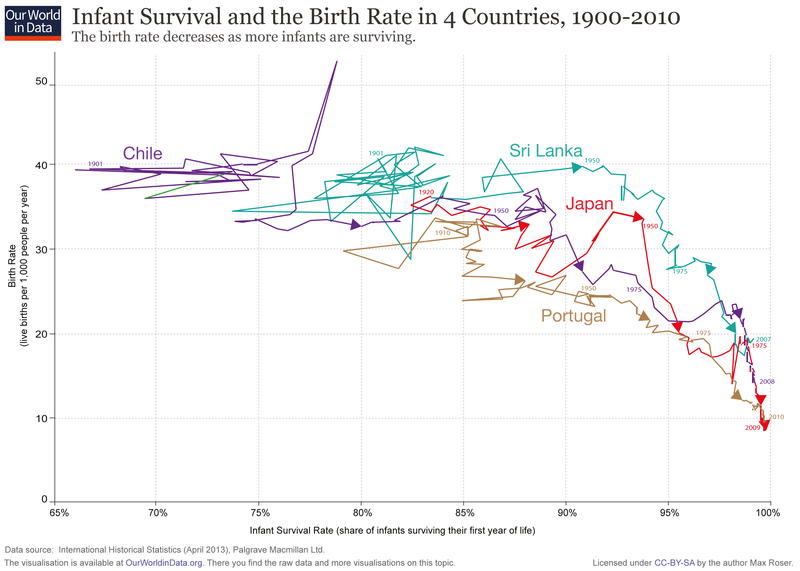Many people think that lower child mortality causes fertility to decline.

One prominent theory for this relationship, as described by Our World in Data1, is that “infant survival reduces the parents’ demand for children”2. (Infants are children under 1 years old).

In this article, I want to look at how we can precisify that theory, and what magnitude the effect could possibly take. What fraction of the decline in birth rates could the theory explain?

Important. I don’t want to make claims here about how parents actually make fertility choices. I only want to examine the implications of various models, and specifically how much of the observed changes in fertility the models could explain.

## Constant number of children

One natural interpretation of “increasing infant survival reduces the parents’ demand for children” is that parents are adjusting the number of births to keep the number of surviving children constant.

Looking at Our World in Data’s graph, we can see that in most of the countries depicted, the infant survival rate went from about 80% to essentially 100%. This is a factor of 1.25. Meanwhile, there were 1/3 as many births. If parents were adjusting the number of births to keep the number of surviving children constant, the decline in infant mortality would explain a change in births by a factor of 1/1.25=0.8, a -0.2 change that is only 30% of the -2/3 change in births.

The basic mathematical reason this happens is that even when mortality is tragically high, the survival rate is still thankfully much closer to 1 than to 0, so even a very large proportional fall in mortality will only amount to a small proportional increase in survival.

Some children survive infancy but die later in childhood. Although Our World in Data’s quote focuses on infant mortality, it makes sense to consider older children too. I’ll look at under-5 mortality, which generally has better data than older age groups, and also captures a large fraction of all child mortality3.

### England (1861-1951)

England is a country with an early demographic transition and good data available.

Doepke 2005 quotes the following numbers:

1861 1951
Infant mortality 16% 3%
1-5yo mortality 13% 0.5%
0-5 yo mortality 27% 3.5%
Survival to 5 years 73% 96.5%
Fertility 4.9 2.1

Fertility fell by 57%, while survival to 5 years rose by 32%. Hence, if parents aim to keep the number of surviving children constant, the change in child survival can explain 43%4 of the actual fall in fertility. (It would have explained only 23% had we erroneously considered only the change in infant survival.)

### Sub-Saharan Africa (1990-2017)

If we look now at sub-Saharan Africa data from the World Bank, the 1990-2017 change in fertility is from 6.3 to 4.8, a 25% decrease, whereas the 5-year survival rate went from 0.82 to 0.92, a 12% increase. So the fraction of the actual change in fertility that could be explained by the survival rate is 44%. (This would have been 23% had we looked only at infant survival).

Source data and calculations. Chart not showing up? Go to the .svg file.

So far, we have seen that this very simple theory of parental decision-making can explain 30-44% of the decline in fertility, while also noticing that considering childhood mortality beyond infancy was important to giving the theory its full due.

However, in more sophisticated models of fertility choices, the theory looks worse.

## A more sophisticated model of fertility decisions

Let us imagine that instead of holding it constant, parents treat the number of surviving children as one good among many in an optimization problem.

An increase in the child survival rate can be seen as a decrease in the cost of surviving children. Parents will then substitute away from other goods and increase their target number of surviving children. If your child is less likely to die as an infant, you may decide to aim to have more children: the risk of experiencing the loss of a child is lower.5

For a more formal analysis, we can turn to the Barro and Becker (1989) model of fertility. I’ll be giving a simplified version of the presentation in Doepke 2005.

In this model, parents care about their own consumption as well as their number of surviving children. The parents maximise6

$U(c,n) = u(c) + n^\epsilon V$

where

• $$n$$ is the number of surviving children and $$V$$ is the value of a surviving child
• $$\epsilon$$ is a constant $$\in (0,1)$$
• $$u(c)$$ is the part of utility that depends on consumption7

The income of a parent is $$w$$, and there is a cost per birth of $$p$$ and an additional cost of $$q$$ per surviving child8. The parents choose $$b$$, the number of births. $$s$$ is the probability of survival of a child, so that $$n=sb$$.

Consumption is therefore $$c=w-(p+qs)b$$ and the problem becomes $$\max_{b} U = u(w-(p+qs)b) + (sb)^\epsilon V$$

Letting $$b^{*}(s)$$ denote the optimal number of births as a function of $$s$$, what are its properties?

The simplest one is that $$sb^*(s)$$, the number of surviving children, is increasing in $$s$$. This is the substitution effect we described intuitively earlier in this section. This means that if $$s$$ is multiplied by a factor $$x$$ (say 1.25), $$b^*(s)$$ will be multiplied more than $$1/x$$ (more than 0.8).

When we looked at the simplest model, with a constant number of children, we guessed that it could explain 30-44% of the fall in fertility. That number is a strict upper bound on what the current model could explain.

What we really want to know, to answer the original question, is how $$b^*(s)$$ itself depends on $$s$$. To do this, we need to get a little bit more into the relative magnitude of the cost per birth $$p$$ and the additional cost $$q$$ per surviving child. As Doepke writes,

If a major fraction of the total cost of children accrues for every birth, fertility [i.e. $$b^*(s)$$] would tend to increase with the survival probability; the opposite holds if children are expensive only after surviving infancy9.

This tells us that falling mortality could actually cause fertility to increase rather than decrease.10

To go further, we need to plug in actual values for the model parameters. Doepke does this, using numbers that reflect the child mortality situation of England in 1861 and 1951, but also what seem to be some pretty arbitrary assumptions about the parent’s preferences (the shape of $$u$$ and the value of $$\epsilon$$).

With these assumptions, he finds that “the total fertility rate falls from 5.0 (the calibrated target) to 4.2 when mortality rates are lowered to the 1951 level”11, a 16% decrease. This represents is 28% of the actually observed fall in fertility to 2.1.

### Extensions of Barro-Becker model

The paper then considers various extensions of the basic Barro-Becker model to see if they could explain the large decrease in fertility that we observe.

For example, it has been hypothesized that when there is uncertainty about whether a child will survive (hitherto absent from the models), parents want to avoid the possibility of ending up with zero surviving children. They therefore have many children as a precautionary measure. Declining mortality (which reduces uncertainty since survival rates are thankfully greater than 0.5) would have a strong negative impacts on births.

However, Doepke also considers a third model, that incorporates not only stochastic mortality but also sequential fertility choice, where parents may condition their fertility decisions on the observed survival of children that were born previously. The sequential aspect reduces the uncertainty that parents face over the number of surviving children they will end up with.

The stochastic and sequential models make no clear-cut predictions based on theory alone. Using the England numbers, however, Doepke finds a robust conclusion. In the stochastic+sequential model, for almost all reasonable parameter values, the expected number of surviving children still increases with $$s$$ (my emphasis):

To illustrate this point, let us consider the extreme case [where] utility from consumption is close to linear, while risk aversion with regards to the number of surviving children is high. … [W]hen we move (with the same parameters) to the more realistic sequential model, where parents can replace children who die early, … despite the high risk aversion with regards to the number of children, total fertility drops only to 4.0, and net fertility rises to 3.9, just as with the benchmark parameters. … Thus, in the sequential setup the conclusion that mortality decline raises net fertility is robust to different preference specifications, even if we deliberately emphasize the precautionary motive for hoarding children.

So even here, the fall in mortality would only explain 35% of the actually observed change in fertility. It seems that the ability to “replace” children who did not survive in the sequential model is enough to make its predictions pretty similar to the simple Barro-Becker model.

1. The quote in context on Our World in Data’s child mortality page: “the causal link between infant [<1 year old] survival and fertility is established in both directions: Firstly, increasing infant survival reduces the parents’ demand for children. And secondly, a decreasing fertility allows the parents to devote more attention and resources to their children.”

2. As an aside, my impression is that if you asked an average educated person “Why do women in developing countries have more children?”, their first idea would be: “because child mortality is higher”. It’s almost a trope, and I feel that it’s often mentioned pretty glibly, without actually thinking about the decisions and trade-offs faced by the people concerned. That’s just an aside though – the theory clearly has prima facie plausibility, and is also cited in serious places like academia and Our World in Data. It deserves closer examination.

3. It should be possible to conduct the Africa analysis for different ages using IMHE’s more granular data, but it’s a bit more work. (There appears to be no direct data on deaths per birth as opposed to per capita, and data on fertility is contained in a different dataset from the main Global Burden of Disease data.)

4. All things decay. Should this Google Sheets spreadsheet become inaccessible, you can download this .xlsx copy which is stored together with this blog.

5. In this light, we can see that the constant model is not really compatible with parents viewing additional surviving children as a (normal) good. Nor of course is it compatible with viewing children as a bad, for then parents would choose to have 0 children. Instead, it could for example be used to represent parents aiming for a socially normative number of surviving children.

6. I collapse Doepke’s $$\beta$$ and $$V$$ into a single constant $$V$$, since they can be treated as such in Model A, the only model that I will present mathematically in this post.

7. Its actual expression, that I omit from the main presentation for simplicity, is $$u(c)=\frac{c^{1-\sigma}}{1-\sigma}$$, the constant relative risk-aversion utility function.

8. There is nothing in the model that compels us to call $$p$$ the “cost per birth”, this is merely for ease of exposition. The model itself only assumes that there are two periods for each child: in the first period, costing $$p$$ to start, children face a mortality risk; and in the second period, those who survived the first face zero mortality risk and cost $$q$$.

9. Once again, Doepke calls the model’s early period “infancy”, but this is not inherent in the model.

10. It’s difficult to speculate about the relative magnitude of $$p$$ and $$q$$, especially if, departing from Doepke, we make the early period of the model, say, the first 5 years of life. If the first period is only infancy, it seems plausible to me that $$q \gg p$$, but then we also fail to capture any deaths after infancy. On the other hand, extending the early period to 5 incorrectly assumes that parents get no utility from children before they reach the age of 5.

11. The following additional context may be helpful to understand this quote:

The survival parameters are chosen to correspond to the situation in England in 1861 . According to Perston et al. (1972) the infant mortality rate (death rate until first birthday) was $$16 \%$$, while the child mortality rate (death rate between first and fifth birthday) was $$13 \%$$. Accordingly, I set $$s_{i}=0.84$$ and $$s_{y}=0.87$$ in the sequential model, and $$s=s_{i} s_{y}=0.73$$ in the other models. Finally, the altruism factor $$\beta$$ is set in each model to match the total fertility rate, which was $$4.9$$ in 1861 (Chenais 1992). Since fertility choice is discrete in Models B and C, I chose a total fertility rate of $$5.0$$ as the target.

Each model is thus calibrated to reproduce the relationship of fertility and infant and child mortality in 1861 . I now examine how fertility adjusts when mortality rates fall to the level observed in 1951 , which is $$3 \%$$ for infant mortality and $$0.5 \%$$ for child mortality. The results for fertility can be compared to the observed total fertility rate of $$2.1$$ in 1951 .

In Model A (Barro-Becker with continuous fertility choice), the total fertility rate falls from $$5.0$$ (the calibrated target) to $$4.2$$ when mortality rates are lowered to the 1951 level. The expected number of surviving children increases from $$3.7$$ to $$4.0$$. Thus, there is a small decline in total fertility, but (as was to be expected given Proposition 1) an increase in the net fertility rate.

]]>
The special case of the normal likelihood function2021-07-31T00:00:00+00:002021-07-31T00:00:00+00:00https://bayes.net/bayes-normal-likelihoodSummary1: The likelihood function implied by an estimate $$b$$ with standard deviation $$\sigma$$ is the probability density function (PDF) of a $$\mathcal{N}(b,\sigma^2)$$. Though this might sound intuitive, it’s actually a special case. If we don’t firmly grasp that it’s an exception, it can be confusing.

Suppose that a study has the point estimator $$B$$ for the parameter $$\Theta$$. The study results are an estimate $$B=b$$ (typically a regression coefficient), and an estimated standard deviation2 $$\hat{sd}(B)=s$$.

In order to know how to combine this information with a prior over $$\Theta$$ in order to update our beliefs, we need to know what is the likelihood function implied by the study. The likelihood function is the probability of observing the study data $$B=b$$ given different values for $$\Theta$$. It is formed from the probability of the observation that $$B=b$$ conditional on $$\Theta=\theta$$, but viewed and used as a function of $$\theta$$ only3:

$\mathcal{L}: \theta \mapsto P(B =b \mid \Theta = \theta)$

The event “$$B=b$$” is often shortened to just “$$b$$” when the meaning is clear from context, so that the function can be more briefly written $$\mathcal{L}: \theta \mapsto P(b \mid \theta)$$.

So, what is $$\mathcal{L}$$? In a typical regression context, $$B$$ is assumed to be approximately normally distributed around $$\Theta$$, due to the central limit theorem. More precisely, $$\frac{B - \Theta}{sd(B)} \sim \mathcal{N}(0,1)$$, and equivalently $$B\sim \mathcal{N}(\Theta,sd(B)^2)$$.

$$sd(B)$$ is seldom known, and is often replaced with its estimate $$s$$, allowing us to write $$B\sim \mathcal{N}(\Theta,s^2)$$, where only the parameter $$\Theta$$ is unknown4.

We can plug this into the definition of the likelihood function:

$\mathcal{L}: \theta \mapsto P(b\mid \theta)= \text{PDF}_{\mathcal{N}(\theta,s^2)}(b) = {\frac {1}{s\sqrt {2\pi }}}\exp \left(-{\frac {1}{2}}\left({\frac {b-\theta }{s }}\right)^{2} \right)$

We could just leave it at that. $$\mathcal{L}$$ is the function5 above, and that’s all we need to compute the posterior. But a slightly different expression for $$\mathcal{L}$$ is possible. After factoring out the square,

$\mathcal{L}: \theta \mapsto {\frac {1}{s {\sqrt {2\pi }}}}\exp \left(-{\frac {1}{2}} {\frac {(b-\theta)^2 }{s^2 }} \right),$

we make use of the fact that $$(b-\theta)^2 = (\theta-b)^2$$ to rewrite $$\mathcal{L}$$ with the positions of $$\theta$$ and $$b$$ flipped:

$\mathcal{L}: \theta \mapsto {\frac {1}{s {\sqrt {2\pi }}}}\exp \left(-{\frac {1}{2}}\left({\frac {\theta-b }{s }}\right)^{2} \right).$

We then notice that $$\mathcal{L}$$ is none other than

$\mathcal{L}: \theta \mapsto \text{PDF}_{\mathcal{N}(b,s^2)}(\theta)$

So, for all $$b$$ and for all $$\theta$$, $$\mathcal{L}: \theta \mapsto \text{PDF}_{\mathcal{N}(\theta,s^2)}(b) = \text{PDF}_{\mathcal{N}(b,s^2)}(\theta)$$.

The key thing to realise is that this is a special case due to the fact that the functional form of the normal PDF is invariant to substituting $$b$$ and $$\theta$$ for each other. For many other distributions of $$B$$, we cannot apply this procedure.

This special case is worth commenting upon because it has personally lead me astray in the past. I often encountered the case where $$B$$ is normally distributed, and I used the equality above without deriving it and understanding where it comes from. It just had a vaguely intuitive ring to it. I would occasionally slip into thinking it was a more general rule, which always resulted in painful confusion.

To understand the result, let us first illustrate it with a simple numerical example. Suppose we observe an Athenian man $$b=200$$ cm tall. For all $$\theta$$, the likelihood of this observation if Athenian men’s heights followed an $$\mathcal{N}(\theta,10)$$ is the same number as the density of observing an Athenian $$\theta$$ cm tall if Athenian men’s heights followed a $$\mathcal{N}(200,10)$$6.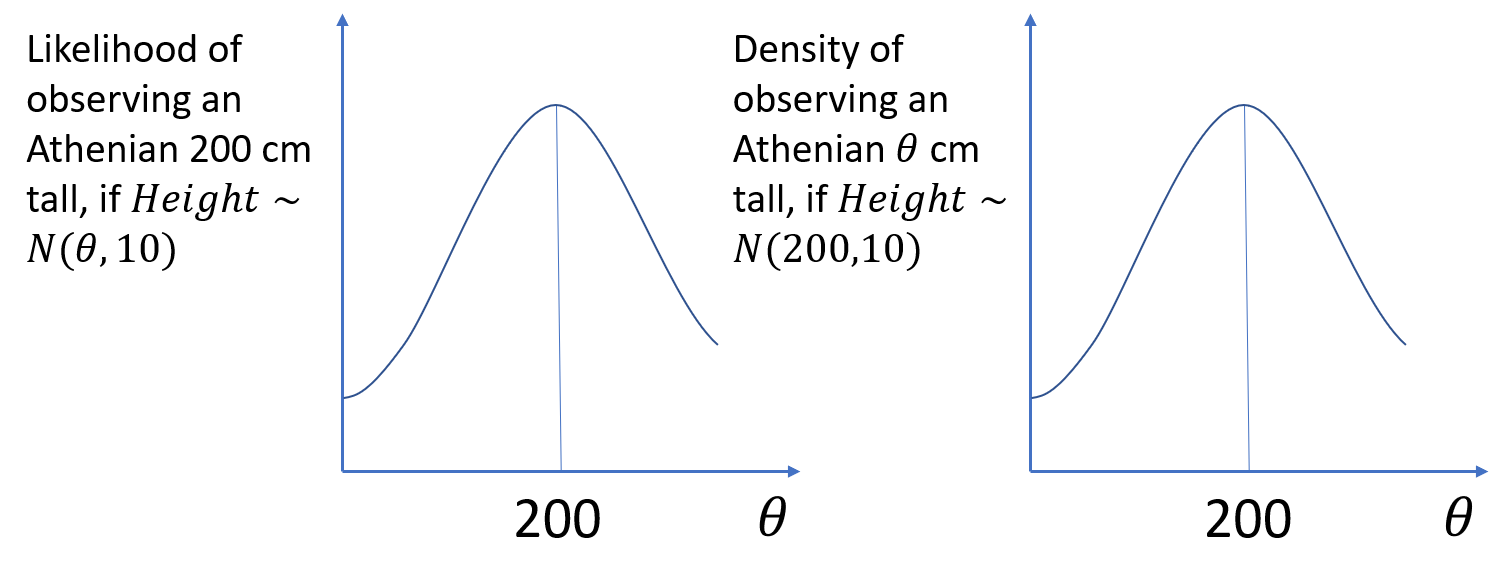Graphical representation of $$\text{PDF}_{\mathcal{N}(\theta,10)}(200) = \text{PDF}_{\mathcal{N}(200,10)}(\theta)$$

When encountering this equivalence, you might, like me, sort of nod along. But puzzlement would be a more appropriate reaction. To compute the likelihood of our 200 cm Athenian under different $$\Theta$$-values, we can substitute a totally different question: “assuming that $$\Theta=200$$, what is the probability of seeing Athenian men of different sizes?”.

The puzzle is, I think, best resolved by viewing it as a special case, an algebraic curiosity that only applies to some distributions. Don’t even try to build an intuition for it, because it does not generalise.

To help understand this better, let’s look at at a case where the procedure cannot be applied.

Suppose for example that $$B$$ is binomially distributed, representing the number of successes among $$n$$ independent trials with success probability $$\Theta$$. We’ll write $$B \sim \text{Bin}(n, \theta)$$.

$$B$$’s probability mass function is

$g: k \mapsto \text{PMF}_{\text{Bin}(n, \theta)}(k) = {n \choose k} \phi^k (1-\phi)^{n-k}$

Meanwhile, the likelihood function for the observation of $$b$$ successes is

$\mathcal{M}: \phi \mapsto \text{PMF}_{\text{Bin}(n, \theta)}(b) = {n \choose b} \phi^b (1-\phi)^{n-b}$

To attempt to take the PMF $$g$$, set its parameter $$\theta$$ equal to $$b$$, and obtain the likelihood function would not just give incorrect values, it would be a domain error. Regardless of how we set its parameters, $$g$$ could never be equal to the likelihood function $$\mathcal{M}$$, because $$g$$ is defined on $$\{0,1,...,n\}$$, whereas $$\mathcal{M}$$ is defined on $$[0,1]$$.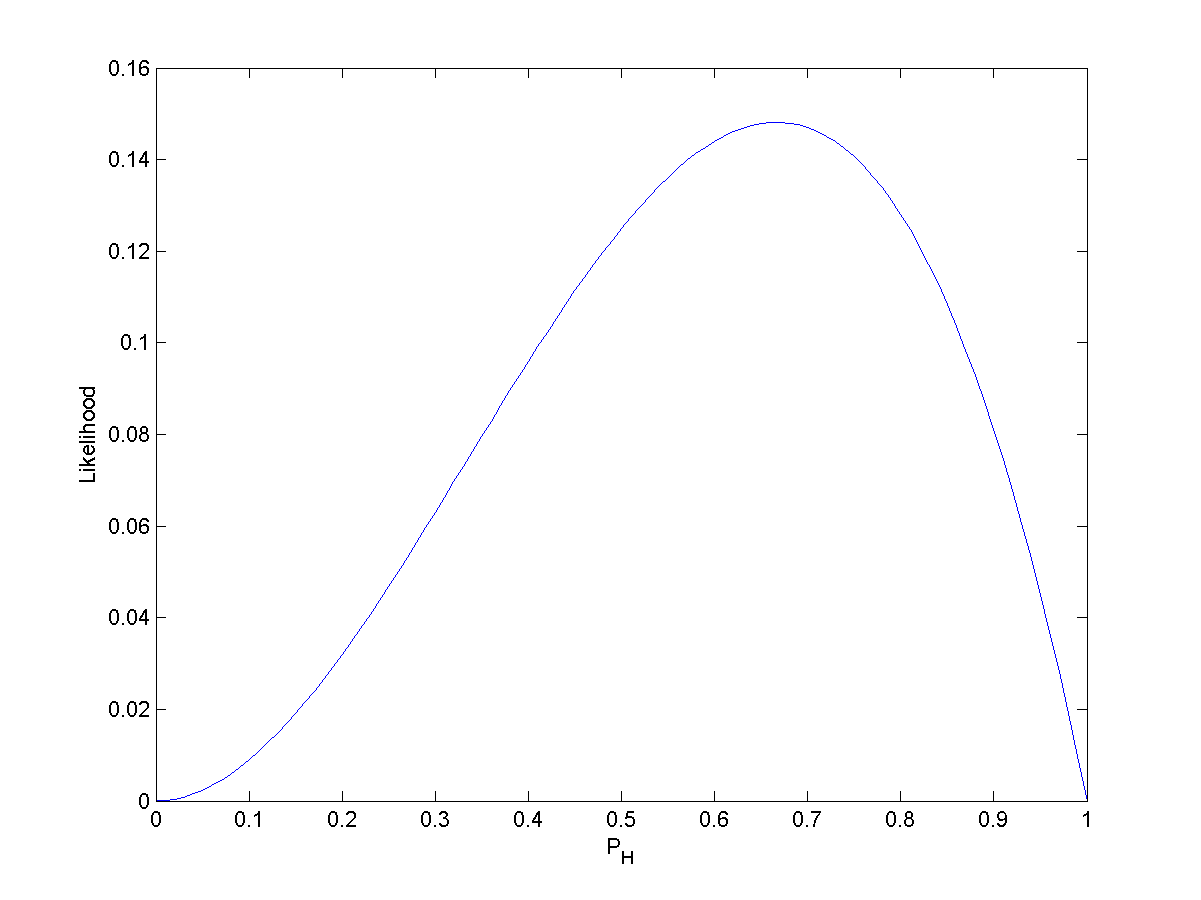The likelihood function $$\mathcal{Q}: P_H \mapsto P_H^2(1-P_H)$$ for the binomial probability of a biased coin landing heads-up, given that we have observed $$\{Heads, Heads, Tails\}$$. It is defined on $$[0,1]$$. (The constant factor $$3 \choose 2$$ is omitted, a common practice with likelihood functions, because these constant factors have no meaning and make no difference to the posterior distribution.)

It’s hopefully now quite intuitive that the case where $$B$$ is normally distributed was a special case.7

Let’s recapitulate.

The likelihood function is the probability of $$b\mid\theta$$ viewed as a function of $$\theta$$ only. It is absolutely not a density of $$\theta$$.

In the special case where $$B$$ is normally distributed, we have the confusing ability of being able to express this function as if it were the density of $$\theta$$ under a distribution that depends on $$b$$.

I think it’s best to think of that ability as an algebraic coincidence, due to the functional form of the normal PDF. We should think of $$\mathcal{L}$$ in the case where $$B$$ is normally distributed as just another likelihood function.

Finally, I’d love to know if there is some way to view this special case as enlightening rather than just a confusing exception.

I believe that to say that a $$\text{PDF}_{\theta,\Gamma}(b)=\text{PDF}_{b,\Gamma}(\theta)$$ (where $$\text{PDF}_{\psi,\Gamma}$$ denotes the PDF of a distribution with one parameter $$\psi$$ that we wish to single out and a vector $$\Gamma$$ of other parameters), is equivalent to saying that the PDF is symmetric around its singled-out parameter. For example, a $$\mathcal{N}(\mu,\sigma^2)$$ is symmetric around its parameter $$\mu$$. But this hasn’t seemed insightful to me. Please write to me if you know an answer to this.

1. Thanks to Gavin Leech and Ben West for feedback on a previous versions of this post.

2. I do not use the confusing term ‘standard error’, which I believe should mean $$sd(B)$$ but is often also used to also denote its estimate $$s$$.

3. I use uppercase letters $$\Theta$$ and $$B$$ to denote random variables, and lower case $$\theta$$ and $$b$$ for particular values (realizations) these random variables could take.

4. A more sophisticated approach would be to let $$sd(B)$$ be another unknown parameter over which we form a prior; we would then update our beliefs jointly about $$\Theta$$ and $$sd(B)$$. See for example Bolstad & Curran (2016), Chapter 17, “Bayesian Inference for Normal with Unknown Mean and Variance”

5. I don’t like the term “likelihood distribution”, I prefer “likelihood function”. In formal parlance, mathematical distributions are a generalization of functions, so it’s arguably technically correct to call any likelihood function a likelihood distribution. But in many contexts, “distribution” is merely used as short for “probability distribution”. So “likelihood distribution” runs the risk of making us think of “likelihood probability distribution” – but the likelihood function is not generally a probability distribution.

6. We are here ignoring any inadequacies of the $$B\sim N(\Theta,s^2)$$ assumption, including but not limited to the fact that one cannot observe men with negative heights.

7. Another simple reminder that the procedure couldn’t possibly work in general is that in general the likelihood function is not even a PDF at all. For example, a broken thermometer that always gives the temperature as 20 degrees has $$P(B=20 \mid \theta) = 1$$ for all $$\theta$$, which evidently does not integrate to 1 over all values of $$\theta$$.

To take a different tack, the fact that the likelihood function is invariant to reparametrization also illustrates that it is not a probability density of $$\theta$$ (thanks to Gavin Leech for the link).

]]>
How to circumvent Sci-Hub ISP block2021-05-15T00:00:00+00:002021-05-15T00:00:00+00:00https://bayes.net/scihub-proxyIn the UK, many internet service providers (ISPs) block Sci-Hub. However, a simple proxy is enough to circumvent this (you don’t even need a VPN). Routing requests through a suitable1 proxy lets you open Sci-Hub in your regular browser as if it weren’t blocked2.

Routing all your traffic through a proxy may come with privacy and security concerns, and will slow your connection a bit. We want to use our proxy only for accessing Sci-Hub.

You can use extensions like ProxySwitchy to tell your browser to automatically use certain proxies, or no proxy at all, for sets of websites that you define.

Unfortunately, this extension, and others like it, require permissions to insert arbitrary JavaScript into any page you visit (the web store accurately explains that the extension can “read and change all your data on the websites you visit”). That’s likely due to insufficiently granular permission definitions by Chrome, and is not the fault of the presumably well-intentioned extension authors. But it freaks me out a little bit (bad things have happened).

Luckily, we can achieve the same effect by writing our own proxy auto-configuration file. A proxy auto-configuration or PAC file contains just a single JavaScript function like this:

function FindProxyForURL (url, host) {

// Sci-Hub requests
if (shExpMatch(host, 'sci-hub.se') || shExpMatch(host,'*.sci-hub.se')) {

// Your proxy address and port number
return 'PROXY 123.456.789:9279';
}

// All other requests
return 'DIRECT';
}


We can instruct the operating system to read this file. Search for instructions on Google (example).

When you use the proxy to access Sci-Hub for the first time in a browser session, the browser will ask you for the username and password to your proxy server. If you’re using Chrome, I’d recommend saving the credentials into the browser’s password manager to avoid having to enter them again.3

There are many free proxies on the Internet, but I find that using the services of an actual for-profit proxy company is well worth it, for the greater speed and reliability. Currently webshare.io (referral link) offers 1 GB per month free, which is quite a lot of Sci-Hub PDFs. After that you can get 250 GB for $2.99 per month.4 ### Step by step instructions 1. Create an account on webshare.io (referral link) 2. Choose a proxy from your list, and copy its address and port number into your PAC file, following the pattern above. 3. Set your operating system to read its proxy settings from this PAC file5. Instructions for this are easy to Google (example). 4. Open Sci-Hub in your browser. Enter your proxy username and password and optionally save these credentials in the browser. You can find the credentials in your webshare.io account. 5. Don’t forget to only use Sci-Hub to look at really old papers that have lapsed into the public domain :) 1. Obviously, the proxy must not itself be on a network that blocks Sci-Hub. I have not come across any proxy that blocks Sci-Hub in this way. 2. Changing your DNS resolver to a public one like Google’s instead of your ISP’s is not sufficient as of 2021, for two ISPs I’ve tested, and I suspect for all UK ISPs that implement blocking. (Many people believe changing the DNS resolver is sufficient. Probably ISPs used to implement simple DNS level blocking and have recently upped their game.) My guess is that instead of merely blocking the request to resolve sci-hub.se at the DNS resolver level, the ISPs are also doing a reverse lookup on every requested IP address to check whether it corresponds to a blacklisted domain. 3. You want to use the Chrome password manager because third-party password managers such as 1Password are not able to auto-fill credentials when logging in to a proxy server (as opposed to logging into a webpage). Note that if you have a third-party password manager extension installed this will disable the browser setting “Offer to save passwords”. I recommend that you temporarily disable your password manager extension, log in to your proxy server, save the password into Chrome, and then enable the extension again. 4. Their home page exemplifies a dark pattern by not showing the pricing by the GB; it just says you get ‘up to unlimited’ bandwidth. You’ll be able to see the actual pricing after you create an account. 5. One gotcha is that Windows 10 forces you to call your PAC file from a web server; it cannot be a local file (??!). To work around this, you can upload your file as a Gist and link to the /raw ]]> Modified respirator to shield myself and others from COVID2021-01-02T00:00:00+00:002021-01-02T00:00:00+00:00https://bayes.net/covid-respiratorSummary: I have tried many types of masks and respirators during the 2020 pandemic. My recommendation is to use ‘elastomeric’ respirators common in industry, and to either filter or completely block off their exhalation valve. The result is a comfortable respirator that I believe offers a high level of protection against airborne diseases to myself and others. I am not an infectious disease expert. # Contents # Elastomeric respirators The effectiveness of a mask can be broken down into two parts: how well the mask fits on your face, and the filtration efficiency of the mask. A further important consideration is comfort. Surgical and cloth masks are comfortable but have poor fit and filtration efficiency. I believe it’s possible to do much better. Respirators that meet the NIOSH N95 or N99 standards for filtration efficiency, such as the N95 respirators pictured below, are popular in healthcare settings.3M N95 respirator (left), N95 respirator in a medical setting (right) In my experience, these have two main downsides: • They may be scarce during pandemics. You should probably leave limited supplies for healthcare workers. • The tight elastic band that ensures a good fit also makes the respirators very uncomfortable for extended use. KN95 and KF95 are respectively the Chinese and Korean-manufactured masks that claim to have the same efficacy as N95s. They come with ear loops rather than behind-the-head elastic bands, so they have a far looser seal than N95s. I suppose you could add your own elastic bands to them to improve the seal, but then they would be just as uncomfortable as N95s. Therefore, they are not a competitive option.A KN95 mask There also exist N95+ masks designed for industrial tasks that produce harmful airborne particles (such as welding or paint spraying). They are called elastomeric respirators, or sometimes industrial respirators.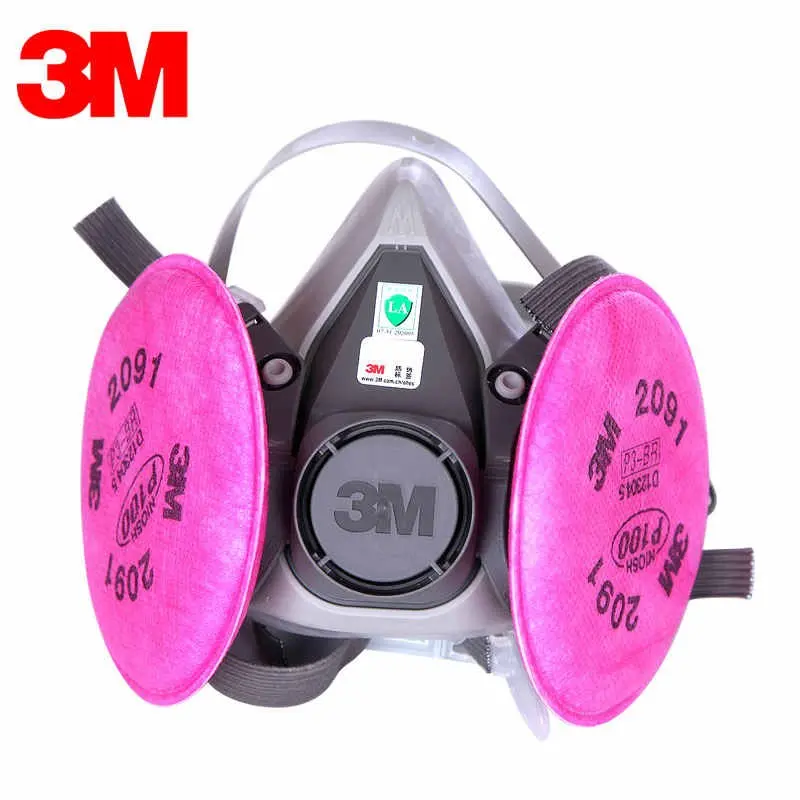High filtration efficiency elastomeric respirators for industrial use. Miller LPR-100 (left), 3M 6200 (right). Compared to healthcare N95s, these respirators: • are more widely available • achieve superior fit by using elastomers shaped like a human face (there is no need to bend a metal nose bridge) • are much more comfortable, mainly because they: • spread the pressure onto a wider area of skin • come in multiple sizes • have adjustable straps • won’t fog up your glasses A downside is that it’s more difficult to be audible through an elastomeric respirator than through an N95 or surgical mask. I am able to be understood by raising my voice, but smooth social interactions are not guaranteed. It’s probably not a great setup for spending time with your friends; you can use a KN95 for that. The fatal flaw1 of these elastomerics when it comes to disease control is that they have an exhalation valve that allows unfiltered air to exit the mask. In PPE jargon, they do not provide source control. (This may be about to change in 2021, see this footnote2. I will try to keep this post updated.) The exhalation valve opening on the Miller LPR-100 We can modify these respirators to filter their exhalation valve (recommendaton A), or completely close it off (recommendation B). (If infection through the mucosal lining of the eyes is an important concern to you, and you don’t wear glasses, you should also wear safety googgles.) # CDC recommendation for exhalation valves During the 2020 pandemic, the US CDC issued the following recommendation, in a blog post from August 8 20203: If only a respirator with an exhalation valve is available and source control is needed, cover the exhalation valve with a surgical mask, procedure mask, or a cloth face covering that does not interfere with the respirator fit. # Recommendation A: 3M 6500QL Series with KN95 and surgical mask If you want to follow something similar to CDC guidance, I recommend: • A 3M 6500QL series respirator • A part of a KN95/KF95 mask tightly covering the exhalation valve3M 6502QL. Unmodified (left), KN95 material covering valve (middle and right) You’ll likely want to add a surgical mask on top of that: • as a backup • for the very small amount of additional filtration it provides • to avoid misunderstandings with strangers3M 6502QL with KN95 material covering valve and a surgical mask on top Surgical masks are not primarily designed to filter aerosols4. It seems clear to me that KN95s and KF95s are superior to a surgical mask for covering an exhalation valve (let a alone a cloth mask). (There is a list of such respirators that have received an emergency use authorization from the FDA. There are probably many low-quality masks fraudulently marketed as KN95 and KF95 at the moment, so make sure you buy from an approved manufacturer.) In the models I have seen, the material in KN95s is far more flexible than in N95s, making allowing you to shape it so that it tightly covers an exhalation valve. It’s slightly fiddly but definitely possible with a bit of dexterity and perseverance. Using a thinner surgical mask would be easier; but the KN95’s extra protection for third parties is well worth it. Here are the steps you should follow (see video): • cut a KN95 in half along the fold • cut one half to size further • use a rubber band and tape to attach the material over the respirator valve. This is better explained with a video than in words. The main thing to know is that you should use the two small ridges in the plastic below the valve to secure the rubber band. • add tape on the upper end of the KN95 material Instructions Unfortunately, for any valve covering approach, there is a trade-off between a fit and the surface area usable for exhale filtration. I have not been able to achieve a good fit when placing a KN95 or surgical mask more loosely over the valve, which would give more surface area. In my setup a small rectangle of KN95 has to do all the filtration, which likely lowers the efficiency. However, the N95 specification is for a flow rate of 85 liters per minute, which is many times the 6 liters per minute breathed by an individual at rest5, so I am not very concerned.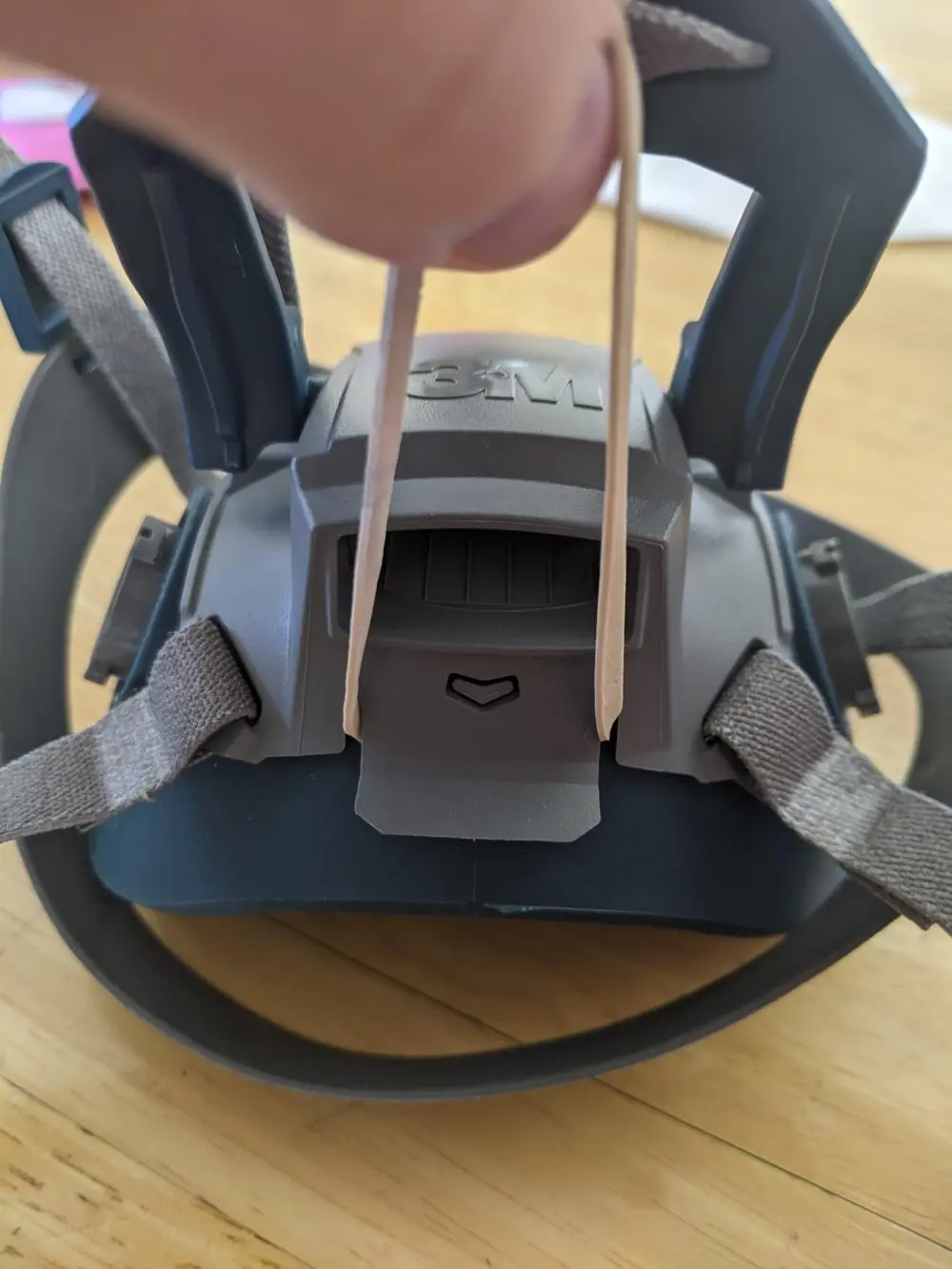Ridges on 6502QL. Note that in the real setup the KN95 will go below the elastic band.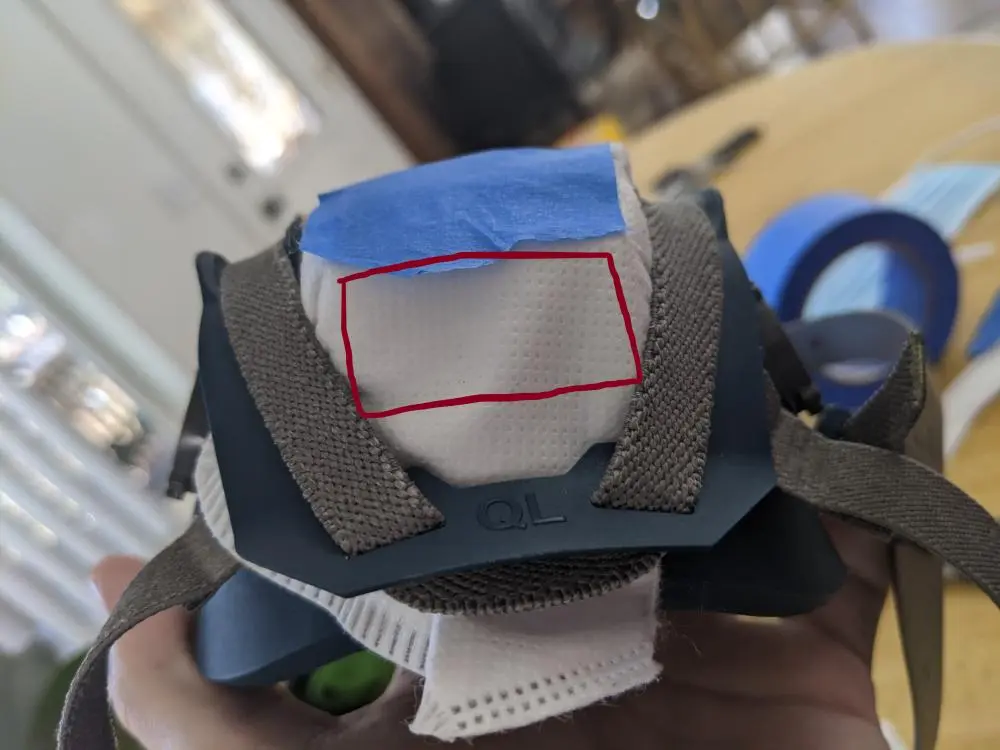Location of the valve underneath the KN95 material. View form below the respirator. ## Choice of respirator I have tried two industrial respirator models, the 3M 6502QL and the Miller LPR-100. I prefer the build quality and aesthetics of the Miller (see below), but its shape makes it almost impossible to get a good seal if you attempt to cover the valve with a surgical mask or KN95. So for this technique, I recommend the 3M 6500QL series. I am aware of three 3M half-facepiece reusable respirator groups, the 6000 series, the 6500 series, and the 7500 series.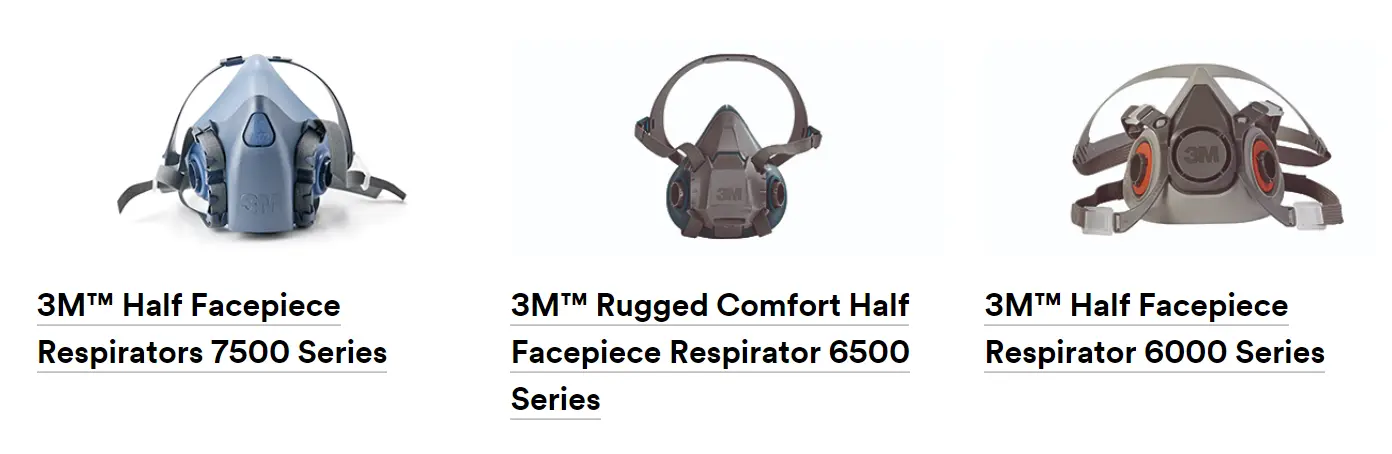3M half-facepiece reusable respirators (3M.com) Since I have only tried a respirator of the 6500 series, I do not have a strong view on which is preferable. I would recommend the 6500, mostly because I have already demonstrated that it’s possible to cover the valve. The 6000 series does not have a downward-facing exhalation valve and may be harder to work with. I’m agnostic about the relative merits of the 7500. The 6500 series has a quick latch version (difference explained here), which is the one I used. I’d recommend the quick latch 6500QL series, because it seems that the latch makes the fit of the KN95 material to the respirator more secure (see video). By the way, attaching a mask on top of the valve makes the quick-latch mechanism much less effective; I never use it. Each series comes in three sizes, large, medium and small. I am a male with a medium-to-large head, and I use a medium (the 6502QL). Regarding whether airlines will accept this setup, I have heard both some positive anecdotes and one negative anecdote. ## Choice of filters for a 3M respirator I use the 3M 2097 P100 filters. You should use lightweight filters that are rated N100, R100 or P100. The “100” means that 99.97% of particles smaller than 0.3 micrometres are filtered out. The letters N, R and P refer to whether the filter is still effective when exposed to oil-based aerosols, this should be irrelevant for our purposes. The weight of the filters is a crucial determinant of comfort. I originally used the 3M respirator with the 3M 60926 cartridges, which filter gases and vapors as well as particles. This was a mistake, as filtering gases and vapors is irrelevant from the point of view of infectious disease, and these cartridges are much heavier than the 3M 2097 P100 filters. Switching to the lighter filters made a world of difference; now wearing the 3M doesn’t bother me at all.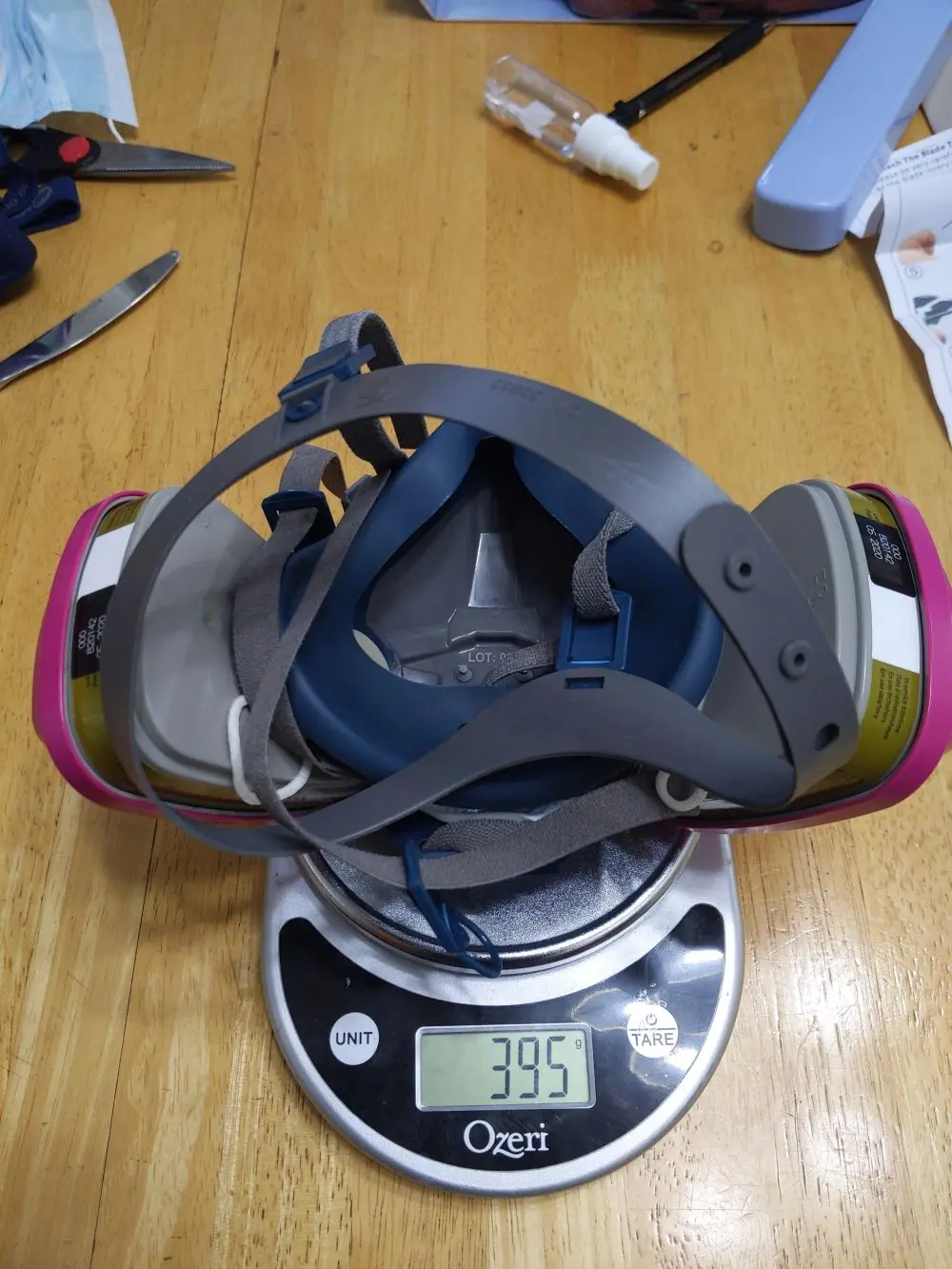The 3M 6502QL respirator weighs 395 g. with 3M 60926 cartridges, but only 128 g. with 3M 2097 filters, a 68% reduction. # Recommmendation B: Miller LPR-100 with tape and surgical mask I believe that, in expectation, the previous method offers slightly worse protection to third parties than a well-fit valveless medical N95, because our makeshift exhalation valve filter may not be entirely effective. This section details another technique which may be able to achieve the best of both worlds: the comfort and availability of industrial masks, and the third-party protection offered by valveless masks. ## How we need to modify the respirator Let’s look at how the valves in industrial masks work. I’ll be using the Miller LPR-100, but the 3M is built similarly. The exhalation valve is at the front. There are also two inhalation valves, one on each side between the mouth and the filter. These only allow air to come into the mask from outside, forcing all the exhaled air to go through the exhalation valve (instead of some of it going back through the filter).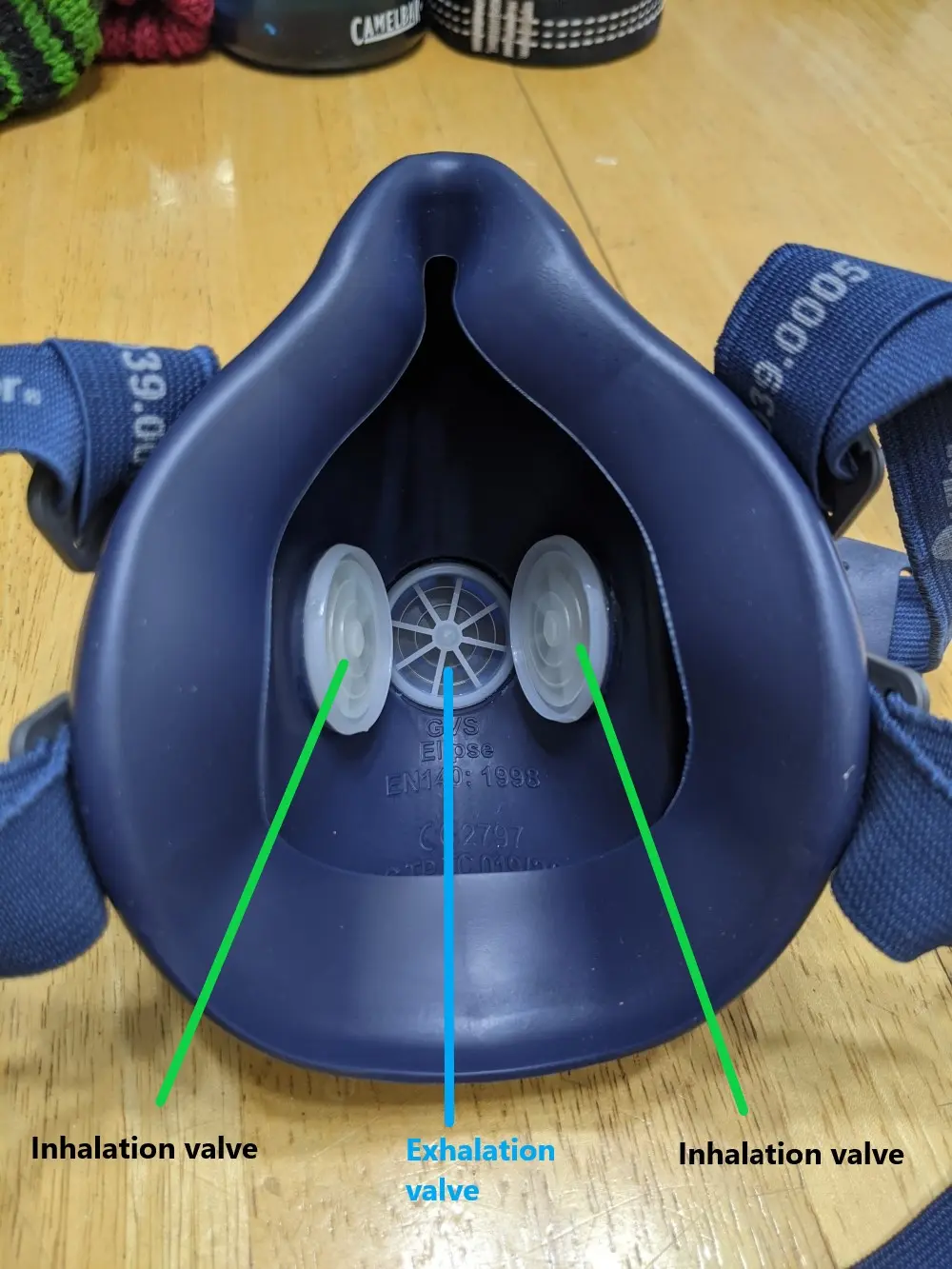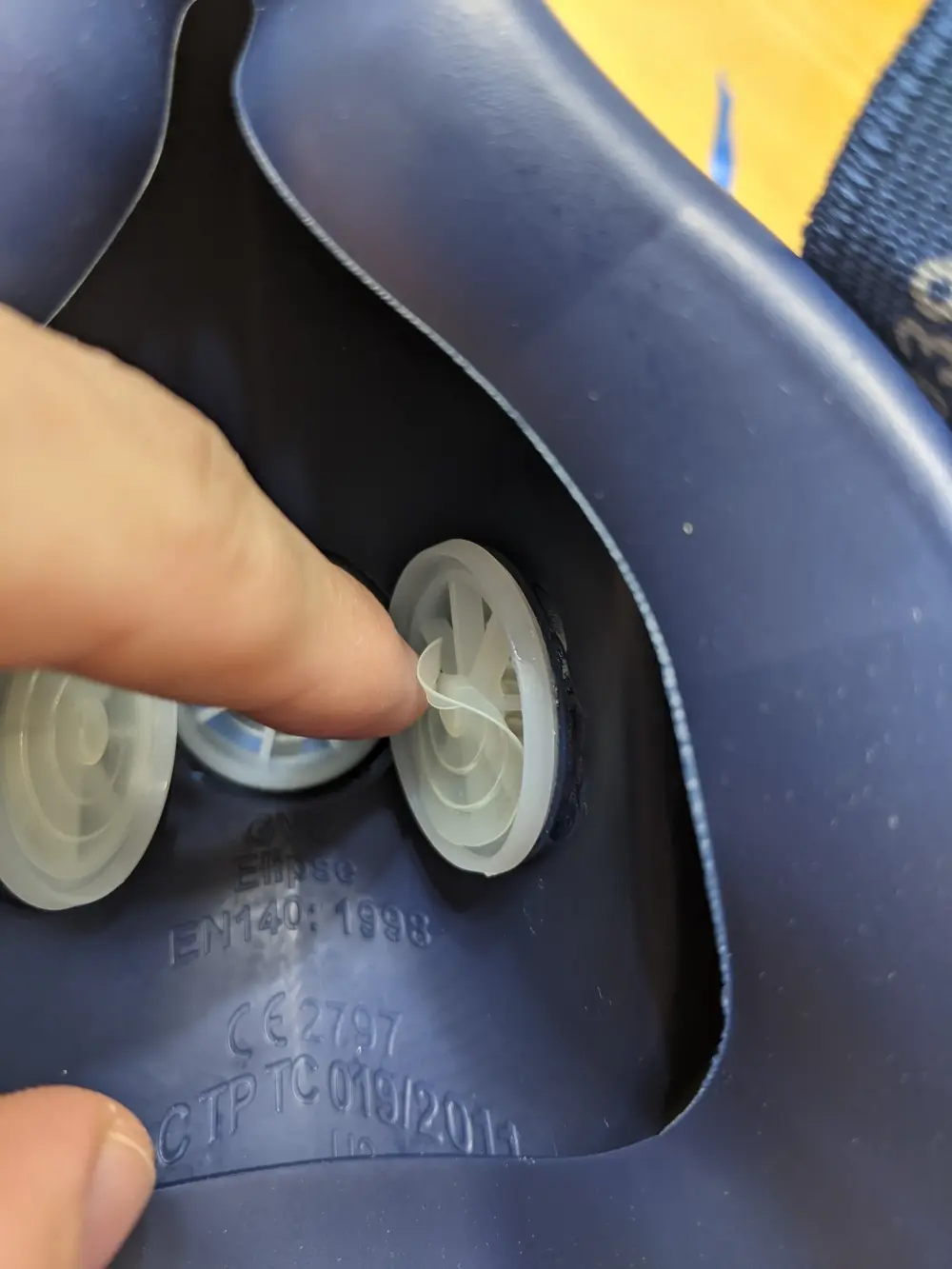Miller LPR-100 valves We need to disable both the inhalation and exhalation valves: • The unfiltered exhalation valve should be completely sealed off. • In order to allow the user to exhale, the inhalation valves need to be turned into simple holes that allow two-way air circulation. This will mean that both inhaled and exhaled air will go through the P100 filters. ## Exhalation valve We can seal off the exhalation valve from the outside with tape6. On the Miller respirator, there is a little plastic cage covering the valve, and this cage can be taped over. Note that tape sticks very poorly to the elastomer (the dark blue material on the Miller). This is why I only place tape on the plastic; this seems to be sufficient.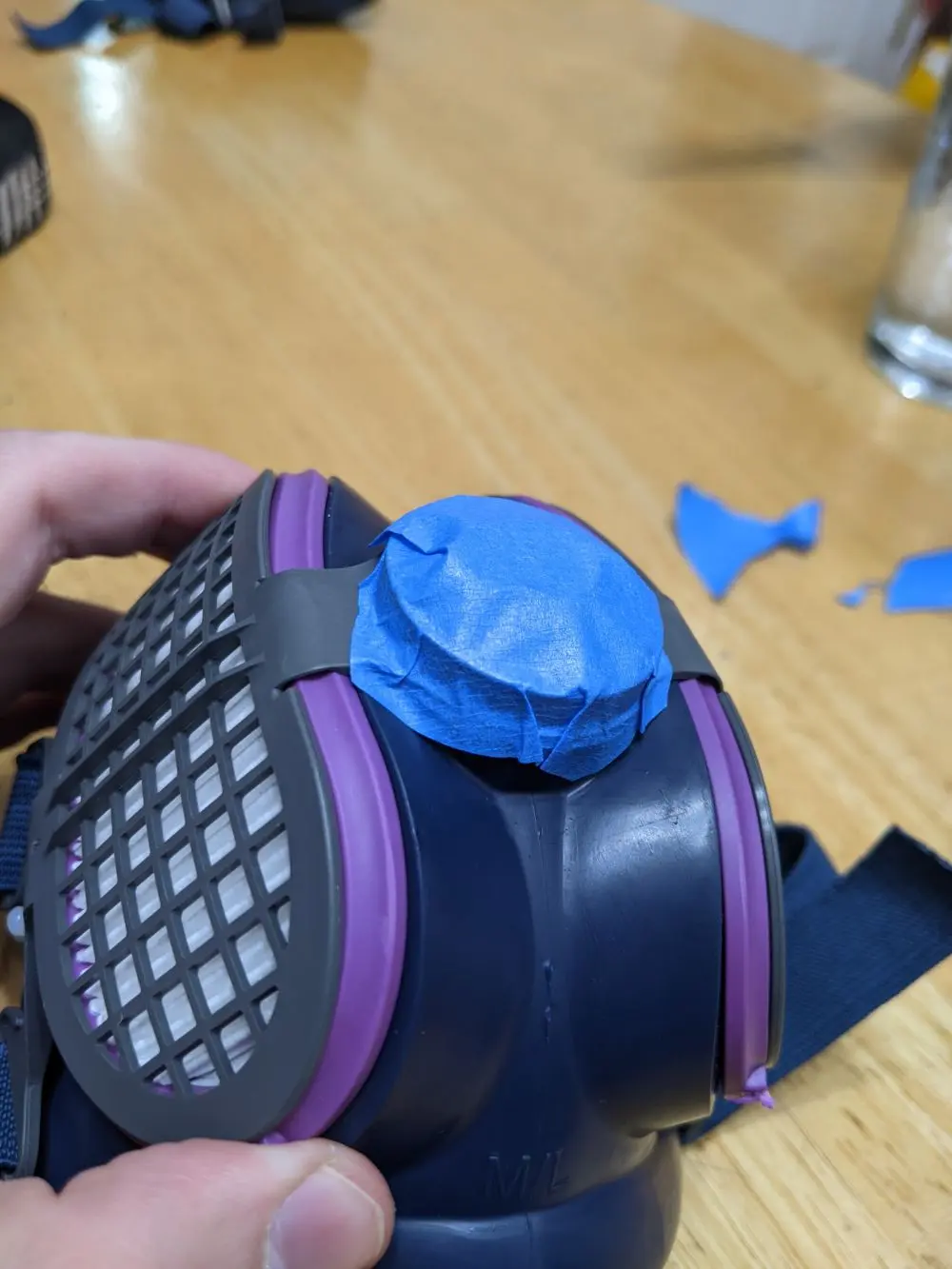Tape on exhalation cage I am using painter’s tape because it’s supposed to pull off without leaving a residue of glue. It’s possible that it would be better to use tape with a stronger adhesive. (A friend of mine commented: “Some ideas for sealing off the exhalation valve: (1) Butyl tape/self-vulcanizing tape. Not so much a sticky tape as a ribbon of moldable putty, so no adhesive residue. This stuff is pretty much unparalleled if you need to make a fully gas- and watertight seal around an irregularly shaped opening in a pinch without making a mess. The fact that it has no adhesive does put some constraints on the geometry of the part you’re sealing off, but I think it would work (better than painter’s tape, at least) on the Miller. (2) Vinyl tape/electrical tape. It’s relatively water-resistant and can be stretched to some extent. The adhesive also sticks to polymers pretty well (although it does leave a lot of residue after some time, but you can clean that off with a bit of IPA).”) You can check the seal of your tape by pressing the mask onto your face and attempting to exhale (with the inhalation valves intact). Air should only be able to escape through the sides of the mask. ## Inhalation valves The inhalation valves are removable and can be pulled out. They are very thin and feel like they might be about to break when you pull them out, but I have been able to pull four of them out without a problem.Touching a valve (left), a valve after it has been pulled out (right)The two inhalation valves (left), the filter now visible through the holes (right) Pushing the valves back in is easy. The tape can be removed and the valves re-inserted, making my modification fully reversible. ## Also use a surgical mask Even if you’re using the tape technique, I recommend also covering the respirator with a surgical mask, since this has no downsides and might have some benefit. The seal on the exhalation valve might not be perfect and may get worse over time, so an extra layer of filtration, however imperfect, is a good backup. It’s also beneficial because it makes what you’re doing legible to others. You don’t want to explain this weird tape business to strangers, even if it’s for their protection.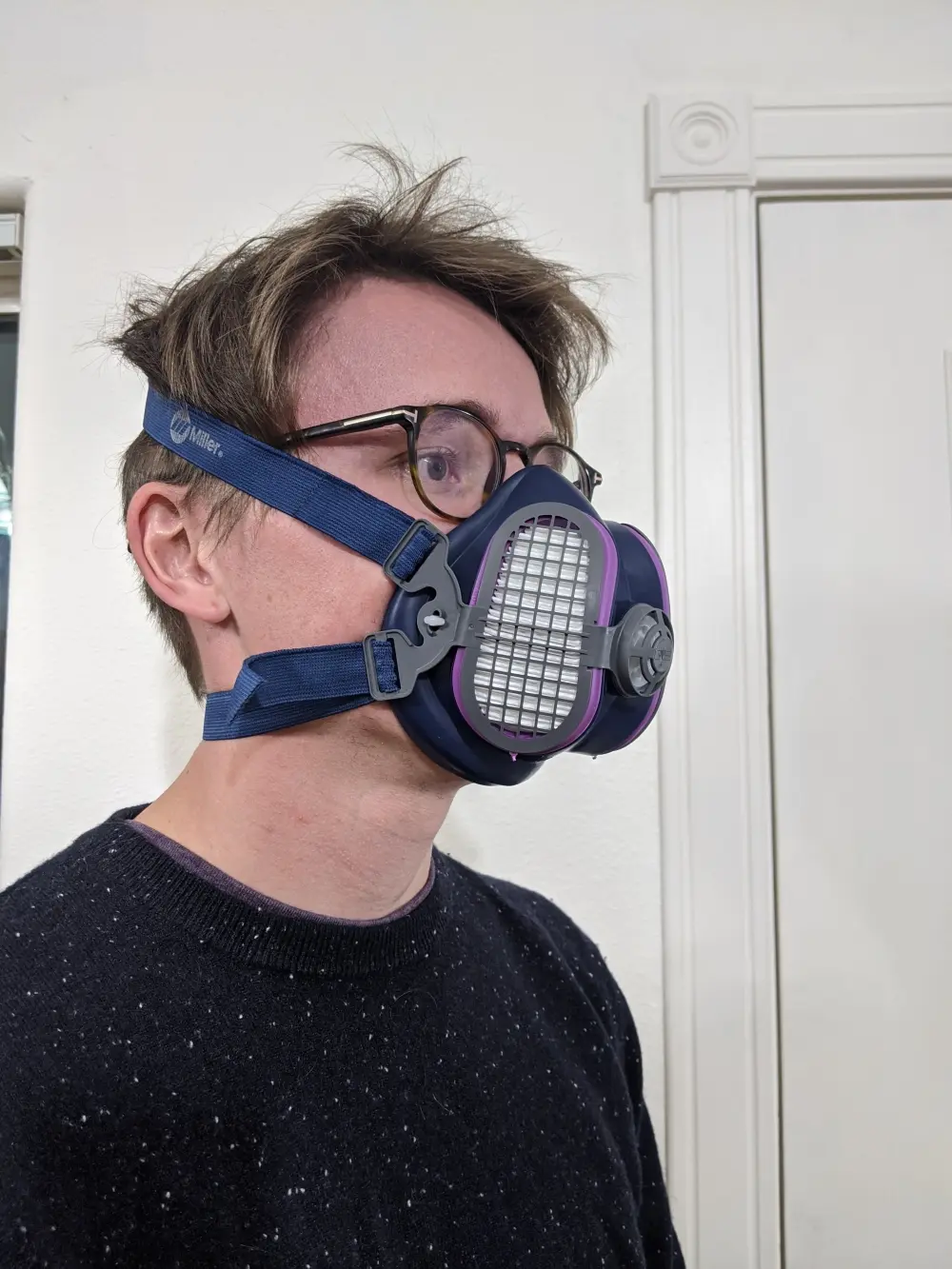Miller LPR-100. Unmodified (left), with tape (middle), with tape and surgical mask (right) ## Choice of respirator For this technique, I recommend the Miller LPR-1007. I recommend the Miller over the 3M because: • its build quality feels superior to me • it looks better • it blocks less of your field of view Since the Miller is better than the 3M, and 3M is such a huge player in this market, I think there’s a decent chance that the Miller is in fact one of the very best options that exists. The Miller weighs 139 g., which is a negligible difference to the 3M’s 128 g I also like the fact that you can buy a neat rigid case to hold the Miller respirator. The case is called the 283374.Miller case, 283374 The Miller model comes with replaceable P100-rated filters, while the 3M can be used with many types of filters and cartridges. If you want to implement this technique on the 3M, it should be possible; all steps will be similar. # Potential concerns The 3M+KN95 method we discussed earlier can be seen as a simple adaptation of CDC guidelines, so I have fewer concerns about it. However, the tape technique involves a more fundamental alteration. This might seem unwise. How do I know I haven’t messed up something crucial, endangering myself and others? Before discussing the specific concerns, it’s useful to consider: what are the relevant alternatives to my recommendation? My best guess is that constantly wearing a correctly fitted medical N95, with the really tight elastic bands, is very slightly safer for others in expectation than the tape method (due to risks of things going wrong, like the tape getting unstuck). However, it is not a likely alternative for everyday use. First, in my experience, N95s are more difficult to fit correctly than industrial masks. Second, for me, these respirators are prohibitively uncomfortable. I have seen few people use them. I think the realistic alternatives for most people are cloth and surgical masks. I am relatively confident that both of my techniques are an improvement on that, for both the user and third parties. By the way, I am not an expert in disease control. I studied economics and philosophy and then worked as a researcher. ## Repeated use Healthcare N95s are supposed to be used only once before being decontaminated. However, I plan to use the same filters many times. Is this a problem? Why are N95s supposed to be used once? According to this CDC guidance, the most significant risk [of extended use and reuse] is of contact transmission from touching the surface of the contaminated respirator. … Respiratory pathogens on the respirator surface can potentially be transferred by touch to the wearer’s hands and thus risk causing infection through subsequent touching of the mucous membranes of the face. … While studies have shown that some respiratory pathogens remain infectious on respirator surfaces for extended periods of time, in microbial transfer [touching the respirator] and reaerosolization [coughing or sneezing through the respirator] studies more than ~99.8% have remained trapped on the respirator after handling or following simulated cough or sneeze. Since I plan to leave the respirator unused for hours or days between each use, and any viral dose on the exterior of the filters is likely to be very small, I don’t think this is a huge concern overall. I am very open to contrary evidence. By the way, based on this guidance, it seems to me we should also worry less about reusing respirators and masks in general, even without decontamination. (Decontamination makes a lot more sense for health care workers who are exposed to COVID patients). It’s good to remember to avoid touching the filters. ## CDC guidelines As explained above, the CDC recommends a surgical or cloth mask to cover the valve. There is no evidence that they considered either of the techniques I described above when issuing their blog post. The tape method is a greater deviation from the CDC guidelines than the KN95-covering method, so if you care about following official guidance you could use the latter. ## Concerns specific to tape method I assign a relatively low chance that the tape method is worse than the CDC recommendation of covering the valve with a surgical mask (my views depend considerably on the tightness of the surgical mask seal). and a very low chance that it’s worse than a surgical mask alone. The probability mass I assign to harm is a combination of concerns about exhaling through the filter reducing its efficacy, and unknown unknowns. ### C02 rebreathing Without the valves, part of the air you inhale will be air that you just exhaled, which contains more C02. I have not personally noticed any effects from this. ### Exhaling through filter Could exhaling through the filter be a bad thing somehow? I wasn’t able to find any source making an explicit statement on this, but I think it’s unlikely to be a problem. One reason to worry is that the founder of Narwall Mask has told me that, according to one filtration expert he spoke to, one-way airflow greatly prolongs the life of the filters compared to two-way airflow. However, based on my small amount of research, I don’t think the life of the filters would be affected to a degree that is practically important. The MSA valveless elastomeric respirator that I mentioned in this footnote2 appears to have filters that can be used for more than 1 month of daily use during the workday; and moreover, we can see in the respirator’s brochure that these filters, with model number 815369, are the same as those that are used in MSA’s line of regular, valved elastomeric respirators (see here). From this I conclude that: two-way airflow through regular P100 filters was considered an acceptable design choice by MSA; and these filters can be used two-way for at least a month of hospital use. In addition, healthcare N95s (without valves) are designed to be exhaled through. They are only rated for a day of use, but I believe this is not because the filter loses efficacy (see section on repeated use). Exhaled air has a relative humidity close to 100%. Could exposure to humid air reduce the efficacy of the filters? In this study of N95 filters, the difference penetration rose from around 2% to around 4% when relative humidity went from 10% to 80%, and this effect increased with duration of continuous use. The flow rate was 85 L/min.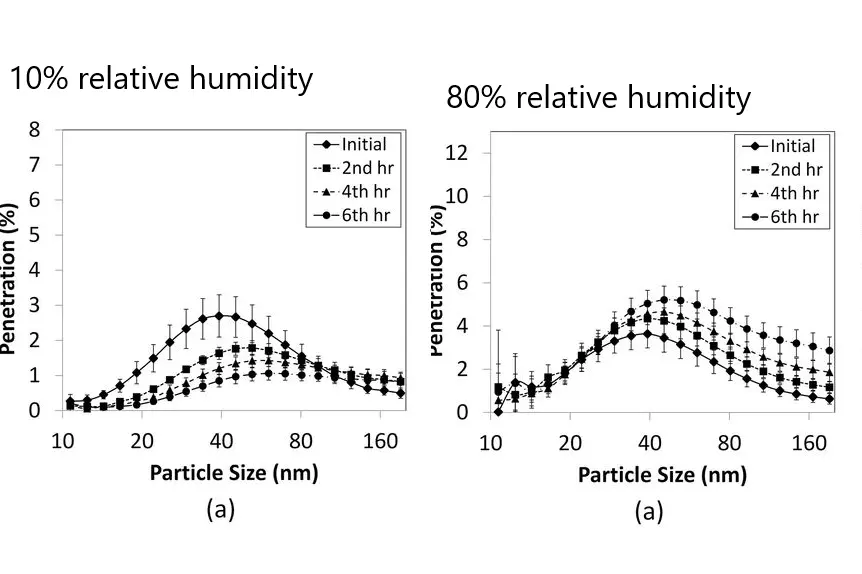Combination of figures 3 and 5, Mahdavi et al. Note that this study, which simulates inhalation of humid air, does not address (except very indirectly) the question of how the exhalation humidity affects the inhalation filtration. ### Discussion of tape technique by others • This NIOSH study tested three modifications of valved respirators: covering the valve on the interior with surgical tape, covering the valve on the interior with an electrocardiogram (ECG) pad, and stretching a surgical mask over the exterior of the respirator. • They found that “penetration was 23% for the masked-over mitigation; penetration was 5% for the taped mitigation; penetration was 2% for the [ECG pad] mitigation”. I would be very interested in more discussion of why the ECG pad did so much better than the surgical tape, the authors don’t say much. One guess could be that the ECG pad has a more powerful adhesive, which would suggest that it’s important to choose a strongly adhesive tape if implementing my technique. • When discussing the choice of modification strategies, the authors wrote that “two concerns are that the adhesive could pull away from the surface, thereby not blocking airflow to the same degree over time, and that these adhesives could contain chemicals that have toxicological effects.”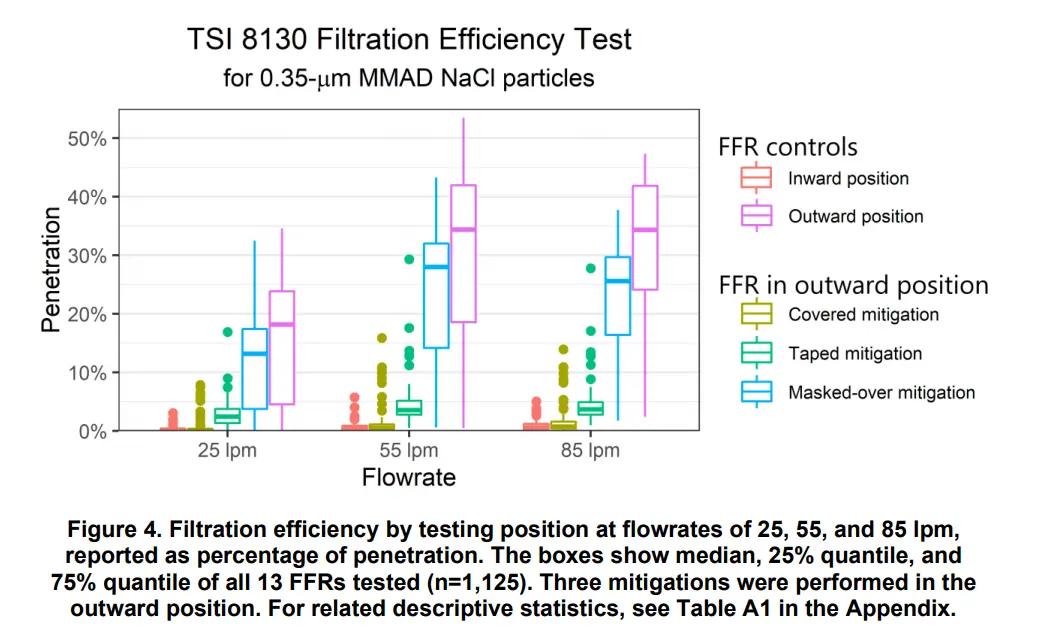• In an FAQ released by 3M, in response to the question of whether one should tape over the exhalation valve, they wrote “3M does not recommend that tape be placed over the exhalation valve”, but do not give any reasons for this beyond the fact that it may become “more difficult to breathe through … if the exhalation valve is taped shut”. • The state of Maine’s Department of Public Safety recommends against tape-covering, but merely because “this would be considered altering the device and violates the manufacturer’s recommendation”. # Overall recommendation I think it’s about 50/50 which of my two methods is better all things considered. They’re close enough that I think the correct decision depends on how much you care about protecting yourself vs source control. If source control is a minor consideration to you, I’d go with the KN95 valve coverage method, otherwise the tape method. (As I said in a previous footnote2, if a valveless elastomeric mask is widely available by the time you read this, that is absolutely a superior option to the hacks I have developed.) (The Narwall Mask is a commercial solution based on a snorkel mask that may be appealing if you don’t mind (i) the lack of NIOSH-approval and (ii) buying from a random startup, and (iii) you don’t mind or even prefer the full-facepiece design.) 1. Or is it fatal? I had always assumed it was a fatal flaw, until I found some experts arguing otherwise. In this commentary, the authors say: “Data characterizing particle release through exhalation valves are presently lacking; it is our opinion that such release will be limited by the complex path particles must navigate through a valve. We expect that fewer respiratory aerosols escape through the exhalation valve than through and around surgical masks, unrated masks, or cloth face coverings, all of which have much less efficient filters and do not fit closely to the face”. I have been able to find some data; this recent NIOSH study finds that valved N95s have 1-40% penetration. “some models … had less than 20% penetration even without any mitigation. Other models … had much greater penetration with a median penetration above 40%.” Note that for these tests, the flow rates of 25-85 L/min are higher than the 6 L/min of a person at rest, and that lower flow rates had lower penetration. Penetration rates of tens of percent are not very good, and not acceptable for my standards, but it’s less bad than I expected, perhaps competitive with surgical masks, and better than cloth masks!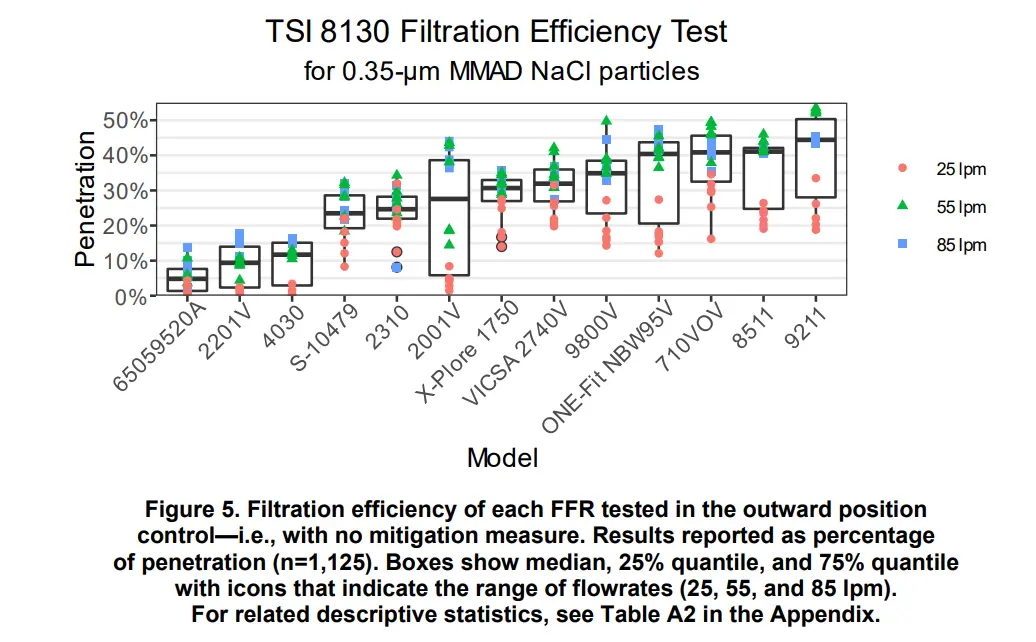2. In fact, as of November 25 2020, the company MSA Safety announced in a press release that the first elastomeric respirator without an exhalation valve has been approved by NIOSH. It’s called the Advantage 290 Respirator. The product page has some good documentation. This journal article from September 2020, although it does not mention MSA, appears to be about the Advantage 290. (This is based on the picture in Fig 1. resembling the picture in the press release, and the fact that the hospitals in the paper are in Pennsylvania and New York states, while MSA is headquartered in Pennsylvania). The article explains how it was rolled out to thousands of healthcare workers (a first wave had 1,840 users). They claim that the cost was “approximately$20 for an elastomeric mask and $10 per cartridge”, which is amazingly low. They write: “After more than 1 month of usage, we have found that filters have not needed to be changed more frequently than once a month”. Unfortunately, it seems to be difficult to get one’s hands on one of these right now. The website invites you to contact sales, and the lowest option for “your budget” is “less than$9,999”.

Moreover, even if you were able to get the Advantage 290, it might be too selfish to do so, since this respirator is likely to otherwise be used by healthcare workers. On the other hand, the price signal you create would in expectation lead to greater quantities being produced, partially offsetting the effect. If you are able to get one by paying a large premium over the hospital price, this may even be net positive for others.

If this respirator became available in large quantities, everything I say here would be obsolete.

By the way, I am astonished that it took until this November 2020 for a PPE company to create a valveless elastomeric respirator, this seems to be a very useful product for any infectious disease situation.  2 3

3. It’s unclear to me how much one should downweight this recommendation due to appearing on a CDC blog rather than as more formal CDC guidance. In the post, the recommendations are called “tips”.

4. The FDA says: “While a surgical mask may be effective in blocking splashes and large-particle droplets, a face mask, by design, does not filter or block very small particles in the air that may be transmitted by coughs, sneezes, or certain medical procedures.”

5. 3M claims that “85 liters per minute (lpm) represents a very high work rate, equivalent to the breathing rate of an individual running at 10 miles an hour”. These lecture notes say that a person has a pulmonary ventilation of 6 L/min at rest, 75 L/min during moderate exercise, and 150L/min during vigorous exercise.

6. I tried two other methods before I settled on using tape: gluing a thin silicon wafer over the valve on the inside of the mask, and applying glue to the valve directly. Both these methods are entirely inferior and should not be used.

7. The model number is ML00895 for the M/L size, and ML00894 for the S/M size.

]]>
Efficient validity checking in monadic predicate logic2020-11-27T00:00:00+00:002020-11-27T00:00:00+00:00https://bayes.net/monadic-predicateMonadic predicate logic (with identity) is decidable. (See Boolos, Burgess, and Jeffrey 2007, Ch. 21. The result goes back to Löwenheim-Skolem 1915).

How can we write a program to check whether a formula is logically valid (and hence also a theorem)?

First, we have to parse the formula, meaning to convert it form a string into a format that represents its syntax in a machine-readable way. That format is an abstract syntax tree like this:

Formula:
∀x(Ax→(Ax∧Bx))

Abstract syntax tree:
∀
├── x
└── →
├── A
│   └── x
└── ∧
├── A
│   └── x
└── B
└── x


Writing the parser was a fun lesson in a fundamental aspect of computer science. But there was nothing novel about this exercise, and not much interesting to say about it.

The focus of this post, instead, is the part of the program that actually checks whether this syntax tree represents a logically valid formula.

To start with, we might try to evaluate the formula under every possible model of a given size. How big does the model need to be?

We can make use of the Löwenheim-Skolem theorem (looking first at the case without identity):

If a sentence of monadic predicate logic (without identity) is satisfiable, then it has a model of size no greater than $$2^k$$, where $$k$$ is the number of predicates in the sentence. (Lemma 21.8 BBJ).

A sentence’s negation is satisfiable if and only if the sentence is not valid, so the theorem equivalently states: a sentence is valid iff it is true under every model of size no greater than $$2^k$$.

For a sentence with $$k$$ predicates, every constant $$c$$ in the model is assigned a list of $$k$$ truth-values, representing for each predicate $$P$$ whether $$P(c)$$. We can use itertools to find every possible such list, i.e. every possible assignment to a constant.

>>> k = 2
>>> possible_predicate_combinations = [i for i in itertools.product([True,False],repeat=k)]
[(True, True), (True, False), (False, True), (False, False)]


The list of every possible assignment to a constant has a length of $$2^k$$.

We can then ask itertools to give us, for a model of size $$m$$, every possible combination of $$m$$ such lists of possible constant-assignments. We let $$m$$ be at most $$2^k$$, because of the theorem.

>>> for m in range(1,2**k+1):
>>>     possible_models = [i for i in itertools.product(possible_predicate_combinations,repeat=m)]
>>>     print(len(possible_models),"possible models of size",m)
>>>     for model in possible_models:
>>>         print(list(model))

4 possible models of size 1
[(True, True)]
[(True, False)]
[(False, True)]
[(False, False)]

16 possible models of size 2
[(True, True), (True, True)]
[(True, True), (True, False)]
[(True, True), (False, True)]
[(True, True), (False, False)]
[(True, False), (True, True)]
[(True, False), (True, False)]
[(True, False), (False, True)]
[(True, False), (False, False)]
[(False, True), (True, True)]
[(False, True), (True, False)]
[(False, True), (False, True)]
[(False, True), (False, False)]
[(False, False), (True, True)]
[(False, False), (True, False)]
[(False, False), (False, True)]
[(False, False), (False, False)]

64 possible models of size 3
[(True, True), (True, True), (True, True)]
[(True, True), (True, True), (True, False)]
[(True, True), (True, True), (False, True)]
[(True, True), (True, True), (False, False)]
[(True, True), (True, False), (True, True)]
[(True, True), (True, False), (True, False)]
[(True, True), (True, False), (False, True)]
[(True, True), (True, False), (False, False)]
[(True, True), (False, True), (True, True)]
[(True, True), (False, True), (True, False)]
...

256 possible models of size 4
[(True, True), (True, True), (True, True), (True, True)]
[(True, True), (True, True), (True, True), (True, False)]
[(True, True), (True, True), (True, True), (False, True)]
[(True, True), (True, True), (True, True), (False, False)]
[(True, True), (True, True), (True, False), (True, True)]
[(True, True), (True, True), (True, False), (True, False)]
[(True, True), (True, True), (True, False), (False, True)]
[(True, True), (True, True), (True, False), (False, False)]
[(True, True), (True, True), (False, True), (True, True)]
[(True, True), (True, True), (False, True), (True, False)]
...


What’s unfortunate here is that for our $$k$$-predicate sentence, we will need to check $$\sum_{m=1}^{2^k} (2^k)^m =\frac{2^k ((2^k)^{2^k} - 1)}{2^k - 1}$$ models. The sum is very roughly equal to its last term, $$(2^k)^{2^k} = 2^{k2^k}$$. For $$k=3$$, this is a number in the billions, for $$k=4$$, it’s a number with 19 zeroes.

So checking every model is computationally impossible in practice. Fortunately, we can do better.

Let’s look back at the Löwenheim-Skolem theorem and try to understand why $$2^k$$ appears in it:

If a sentence of monadic predicate logic (without identity) is satisfiable, then it has a model of size no greater than $$2^k$$ , where $$k$$ is the number of predicates in the sentence. (Lemma 21.8 BBJ).

As we’ve seen, $$2^k$$ is the number of possible combinations of predicates that can be true of a constant in the domain. Visually, this is the number of subsets in a partition of the possibility space: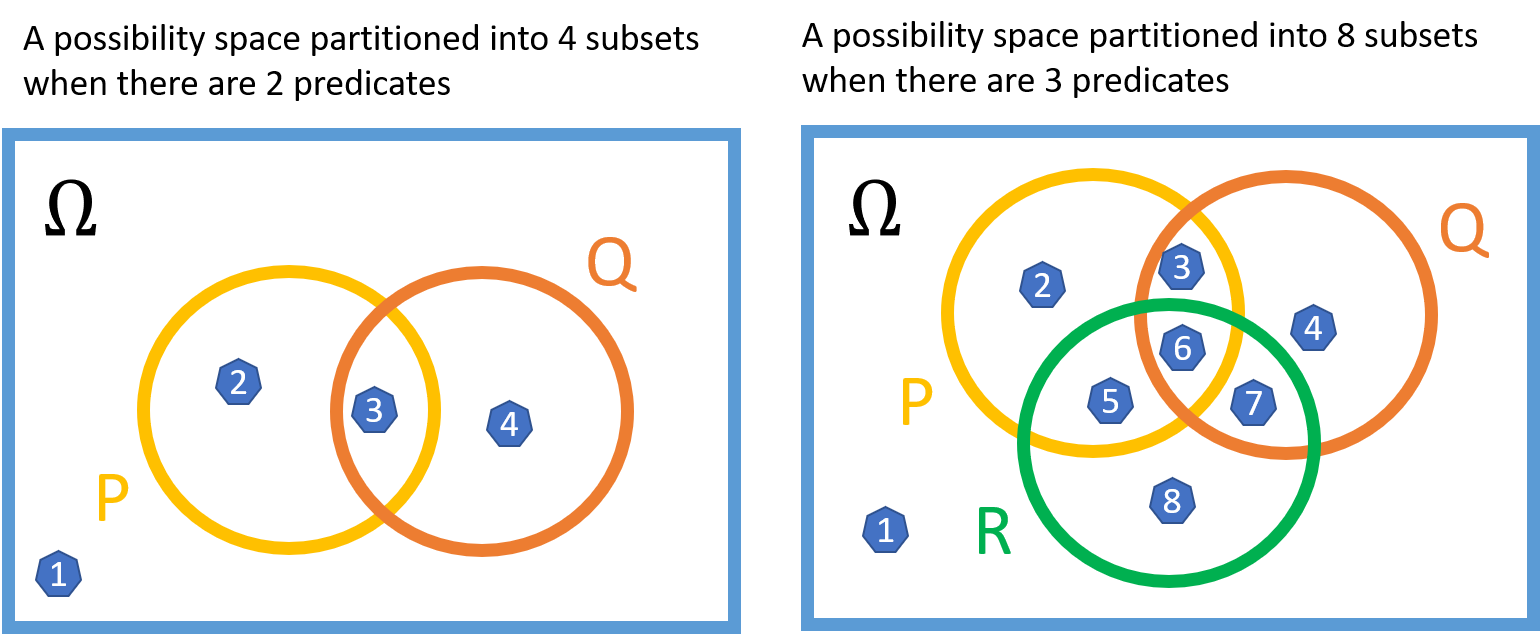If a model had a size of, say, $$2^k + 1$$, one of the subsets in the partition would need to contain more than one element. But this additional element would be superfluous insofar as the truth-value of the sentence is concerned. The partition subset corresponds to a predicate-combination that would already be true with just one element in the subset, and will continue to be true if more elements are added. Take, for example, the subset labeled ‘8’ in the drawing, which corresponds to $$R \land \neg Q \land \neg P$$. The sentence $$\exists x R(x) \land \neg Q(x) \land \neg P(x)$$ is true whether there are one, two, or a million elements in subset 8. Similarly, $$\forall x R(x) \land \neg Q(x) \land \neg P(x)$$ does not depend on the number of elements in subset 8.

Seeing this not only illuminates the theorem, but also let us see that the vast majority of the multitudinous $$\sum_{m=1}^{2^k} (2^k)^m$$ models we considered earlier are equivalent. All that matters for our sentence’s truth-value is whether each of the subsets is empty or non-empty. This means there are in fact only $$2^{(2^k)}-1$$ model equivalence classes to consider. We need to subtract one because the subsets cannot all be empty, since the domain needs to be non-empty.

>>> k = 2
>>> eq_classes = [i for i in itertools.product(['Empty','Non-empty'],repeat=2**k)]
>>> eq_classes.remove(('Empty',)*k**2)
>>> eq_classes
[('Empty', 'Empty', 'Empty', 'Non-empty'),
('Empty', 'Empty', 'Non-empty', 'Empty'),
('Empty', 'Empty', 'Non-empty', 'Non-empty'),
('Empty', 'Non-empty', 'Empty', 'Empty'),
('Empty', 'Non-empty', 'Empty', 'Non-empty'),
('Empty', 'Non-empty', 'Non-empty', 'Empty'),
('Empty', 'Non-empty', 'Non-empty', 'Non-empty'),
('Non-empty', 'Empty', 'Empty', 'Empty'),
('Non-empty', 'Empty', 'Empty', 'Non-empty'),
('Non-empty', 'Empty', 'Non-empty', 'Empty'),
('Non-empty', 'Empty', 'Non-empty', 'Non-empty'),
('Non-empty', 'Non-empty', 'Empty', 'Empty'),
('Non-empty', 'Non-empty', 'Empty', 'Non-empty'),
('Non-empty', 'Non-empty', 'Non-empty', 'Empty'),
('Non-empty', 'Non-empty', 'Non-empty', 'Non-empty')]


We are now ready to consider the extension to monadic predicate logic with identity. With identity, it’s possible to check whether any two members of a model are distinct or identical. This means we can distinguish the case where a partition subset contains one element from the case where it contains several. But we can still only distinguish up to a certain number of elements in a subset. That number is bounded above by the number of variables in the sentence1 (e.g. if you only have two variables $$x$$ and $$y$$, it’s not possible to construct a sentence that asserts there are three different things in some subset). Indeed we have:

If a sentence of monadic predicate logic with identity is satisfiable, then it has a model of size no greater than $$2^k \times r$$, where $$k$$ is the number of monadic predicates and $$r$$ the number of variables in the sentence. (Lemma 21.9 BBJ)

By analogous reasoning to the case without identity, we need only consider $$(r+1)^{(2^k)}-1$$ model equivalence classes. All that matters for our sentence’s truth-value is whether each of the subsets has $$0, 1, 2 ...$$ or $$r$$ elements in it.

>>> k = 2
>>> r = 2
>>> eq_classes = [i for i in itertools.product(range(r+1),repeat=2**k)]
>>> eq_classes.remove((0,)*k**2)
>>> eq_classes
[(0, 0, 0, 1),
(0, 0, 0, 2),
(0, 0, 1, 0),
(0, 0, 1, 1),
(0, 0, 1, 2),
(0, 0, 2, 0),
(0, 0, 2, 1),
(0, 0, 2, 2),
(0, 1, 0, 0),
(0, 1, 0, 1),
(0, 1, 0, 2),
(0, 1, 1, 0),
(0, 1, 1, 1),
...

1. I believe it should be possible to find a tighter bound based on the number of times the equals sign actually appears in the sentence. For example, if equality is only used once, e.g. in $$\exists x \exists y \neg(x =y) \land \phi$$ where $$\phi$$ does not contain equality, it seems clear that the number of variables in $$\phi$$ should have no bearing on the model size that is needed. My hunch is that more generally you need $$n*(n-1)/2$$ uses of ‘$$=$$’ to assert that $$n$$ objects are distinct, so, for example if ‘$$=$$’ appears 5 times you can distinguish 3 objects in a subset, or with 12 ‘$$=$$’s you can distinguish 5 objects. It’s only an intuition and I haven’t checked it carefully.

]]>
Protecting yourself from Vanguard’s poor security practices2020-11-25T00:00:00+00:002020-11-25T00:00:00+00:00https://bayes.net/vanguardThe index fund company Vanguard supports two-factor authentication (2fa) with SMS. SMS is known to be the worst form of 2fa, because it is vulnerable to so-called SIM-swapping attacks. In this type of attack, the malicious party impersonates you and tells your telephone company you’ve lost your SIM card. They request that your number be moved to a new SIM card that they possess. The attacker could call up the company and ask them to activate a spare SIM card that they’ve acquired earlier, or they could visit a store and ask to be given a new SIM card for your number. Then they can receive your security codes.

The security of SMS-based 2fa is only as good as your phone operator’s protections against SIM-swapping, meaning probably not very good. The attacker only needs to convince one mall telco shop employee that they’re you, and they can likely try as many times as they want.

Vanguard claims to also support hardware security keys as a second factor. These are widely regarded as the gold standard for 2fa. Not only are they a true piece of hardware that can’t be SIM-swapped, they also ensure you’re protected even if you get fooled by a phishing attempt (by sending a code that is a function of the URL you are on).

So good news, right? No, because Vanguard made the inexplicable decision to force everyone who uses a security key to also keep SMS 2fa enabled as a fallback option. This utterly defeats the point. The attacker can just click ‘lost security key’ and get an SMS code instead. Users who enable the security key feature actually make their account less secure, because it now has two possible attack surfaces instead of one.People have been complaining about this for years, ever since Vanguard first introduced security keys. On the Bogleheads forum (where intense Vanguard fanatics congregate), this issue was recognized in this thread from 2016, this one from 2017, this one from 2018, and several others. There are plenty of complaints on reddit too. It’s fair to assume some of these people will have contacted Vanguard directly too.

It’s disappointing that a company with over 6 trillion dollars of assets under management offers its clients a security “feature” that makes their accounts less secure.

The workaround I’ve found is to use a Google Voice number to receive SMS 2fa codes (don’t bother with the useless security key). Of course, you must set the Google Voice number not to forward SMS messages to your main phone number, which would defeat the purpose. Then, the messages can only be read by being logged in to the Google account. A Google account can be made into an extremely hardened target. The advanced protection program is available for the sufficiently paranoid.

If you don’t receive the SMS for some reason, you can also receive the authentication code with an automated call to the same number.

You need to have an existing US phone number to create a Google Voice account.

By the way, using Google Voice may not work for all companies that force you to use SMS 2fa. I have verified that it works for Vanguard. This poster claims that “many financial institutions will now only send their 2FA codes to true mobile phone numbers. Google Voice numbers are land lines, with the text messaging function spliced on via a third-party messaging gateway”.

]]>
Eliciting probability distributions from quantiles2020-08-28T00:00:00+00:002020-08-28T00:00:00+00:00https://bayes.net/quantilesWe often have intuitions about the probability distribution of a variable that we would like to translate into a formal specification of a distribution. Transforming our beliefs into a fully specified probability distribution allows us to further manipulate the distribution in useful ways.

For example, you believe that the cost of a medication is a positive number that’s about 10, but with a long right tail: say, a 10% probability of being more than 100. To use this cost estimate in a Monte Carlo simulation, you need to know exactly what distribution to plug in. Or perhaps you have a prior about the effect of creatine on cognitive performance, and you want to formally update that prior using Bayes’ rule when a new study comes out. Or you want to make a forecast about a candidate’s share of the vote and evaluate the accuracy of your forecast using a scoring rule.

In most software, you have to specify a distribution by its parameters, but these parameters are rarely intuitive. The normal distribution’s mean and standard deviation are somewhat intuitive, but this is the exception rather than the rule. The lognormal’s mu and sigma correspond to the mean and standard deviation of the variable’s logarithm, something I personally have no intuitions about. And I don’t expect good results if you ask someone to supply a beta distribution’s alpha and beta shape parameters.

I have built a tool that creates a probability distribution (of a given family) from user-supplied quantiles, sometimes also called percentiles. Quantiles are points on the cumulative distribution function: $$(p,x)$$ pairs such that $$P(X<x)=p$$. To illustrate what quantiles are, we can look at the example distribution below, which has a 50th percentile (or median) of -1 and a 90th percentile of 10.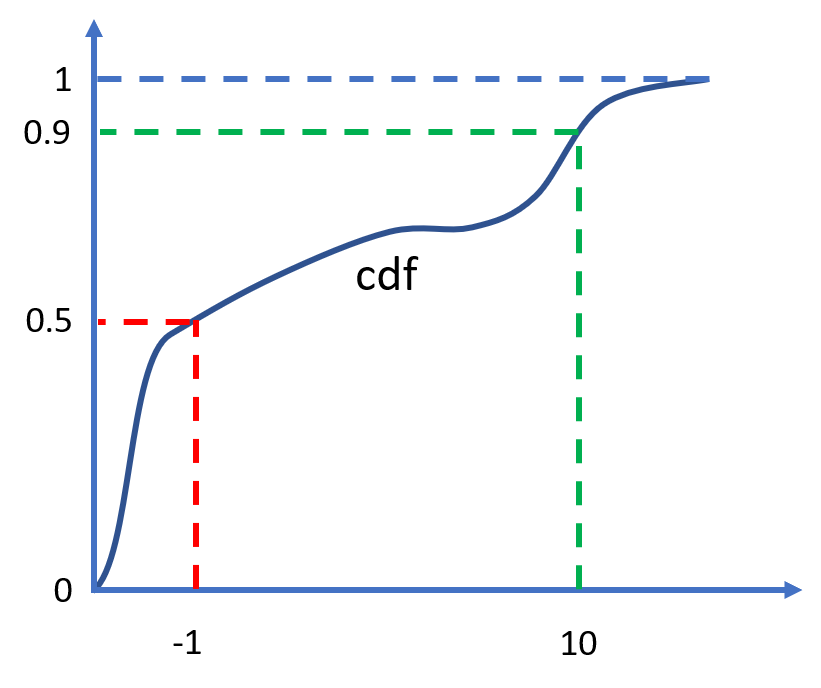A cumulative distribution function with a median of -1 and a 90th percentile of 10

The code is on GitHub, and the webapp is here.

Let’s run through some examples of how you can use this tool. At the end, I will discuss how it compares to other probability elicitation software, and why I think it’s a valuable addition.

The tool supports the normal and lognormal distributions, and more of the usual distribution families could easily be added. The user supplies the distribution family, along with an arbitrary number of quantiles. If more quantiles are provided than the distribution has parameters (more than two in this case), the system is over-determined. The tool then uses least squares to find the best fit.

This is some example input:

family = 'lognormal'
quantiles = [(0.1,50),(0.5,70),(0.75,100),(0.9,150)]


And the corresponding output:

More than two quantiles provided, using least squares fit

Lognormal distribution
mu 4.313122980928514
sigma 0.409687416531683

quantiles:
0.01 28.79055927521217
0.1 44.17183774344628
0.25 56.64439363937313
0.5 74.67332855521319
0.75 98.44056294458953
0.9 126.2366766332274
0.99 193.67827989071688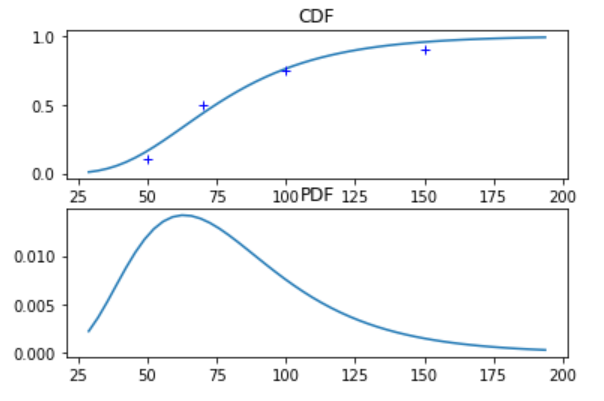# Metalog distribution

The feature I am most excited about, however, is the support for a new type of distribution developed specifically for the purposes of flexible elicitation from quantiles, called the meta-logistic distribution. It was first described in Keelin 2016, which puts it at the cutting edge compared to the venerable normal distribution invented by Gauss and Laplace around 1810. The meta-logistic, or metalog for short, does not use traditional parameters. Instead, it can take on as many terms as the user provides quantiles, and adopts whatever shape is needed to fit these quantiles very closely. Closed-form expressions exist for its quantile function (the inverse of the CDF) and for its PDF. This leads to attractive computational properties (see footnote)1.

Keelin explains that

[t]he metalog distributions provide a convenient way to translate CDF data into smooth, continuous, closed-from distribution functions that can be used for real-time feedback to experts about the implications of their probability assessments.

The metalog quantile function is derived by modifying the logistic quantile function,

$\mu + s \ln{\frac{y}{1-y}} \quad\text{ for } 0 < y < 1$

by letting $$\mu$$ and $$s$$ depend on $$y$$ instead of being constant.

As Keelin writes, given a systematically increasing $$s$$ as one moves from left to right, a right skewed distribution would result. And a systematically decreasing $$\mu$$ as one moves from left to right would make the distribution spikier in the middle with correspondingly heavier tails.

By modifying $$s$$ and $$\mu$$ in ever more complex ways we can make the metalog take on almost any shape. In particular, in most cases the metalog CDF passes through all the provided quantiles exactly2. Moreover, we can specify the metalog to be unbounded, to have arbitrary bounds, or to be semi-bounded above or below.

Instead of thinking about which of several highly constraining distribution families to use, just choose the metalog and let your quantiles speak for themselves. As Keelin says:

one needs a distribution that has flexibility far beyond that of traditional distributions – one that enables “the data to speak for itself” in contrast to imposing unexamined and possibly inappropriate shape constraints on that data.

For example, we can fit an unbounded metalog to the same quantiles as above:

family = 'metalog'
quantiles = [(0.1,50),(0.5,70),(0.75,100),(0.9,150)]
metalog_leftbound = None
metalog_rightbound = None

Meta-logistic distribution

quantiles:
0.01 11.968367580205552
0.1 50.000000000008185
0.25 58.750000000005215
0.5 70.0
0.75 100.00000000000519
0.9 150.00000000002515
0.99 281.7443263650518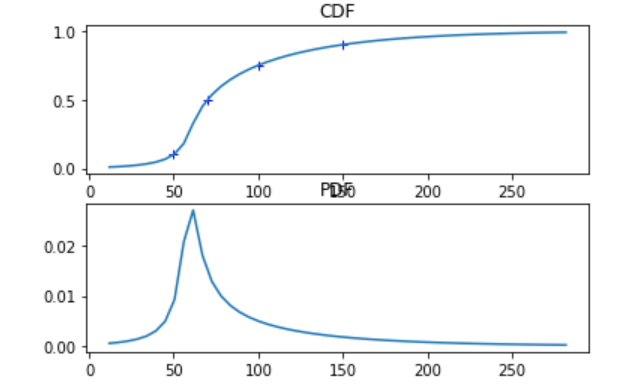The metalog’s actual parameters (as opposed to the user-supplied quantiles) have no simple interpretation and are of no use unless the next piece of software you’re going to use knows what a metalog is. Therefore the program doesn’t return the parameters. Instead, if we want to manipulate this distribution, we can use the expressions of the PDF and CDF that the software provides, or alternatively export a large number of samples into another tool that accepts distributions described by a list of samples (such as the Monte Carlo simulation tool Guesstimate). By default, 5000 samples will be printed; you can copy and paste them.

# Approaches to elicitation

How does this tool compare to other approaches for creating subjective belief distributions? Here are the strategies I’ve seen.

## Belief intervals

The first approach is to provide a belief interval that is mapped to some fixed quantiles, e.g. a 90% belief interval (between the 0.05 and 0.95 quantile) like on Guesstimate. Metaculus provides a graphical way to input the same data, allowing the user to drag the quantiles across a line under a graph of the PDF. This is the simplest and most user-friendly approach. The tool I built incorporates the belief interval approach while going beyond it in two ways. First, you can provide completely arbitrary quantiles, instead of specifically the 0.05 and 0.95 – or some other belief interval symmetric around 0.5. Second, you can provide more than two quantiles, which allows the user to query intuitive information about more parts of the distribution.Guesstimate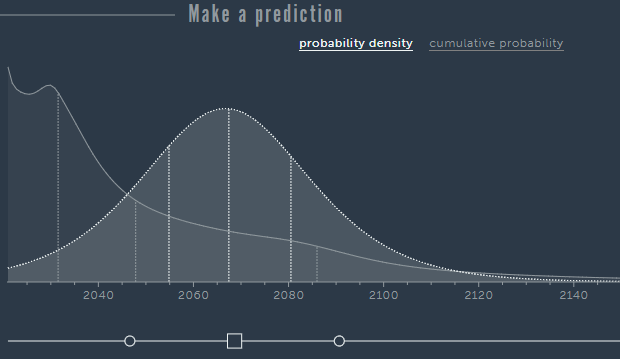Metaculus

## Drawing

Another option is to draw the PDF on a canvas, in free form, using your mouse. This is the very innovative approach of probability.dev.3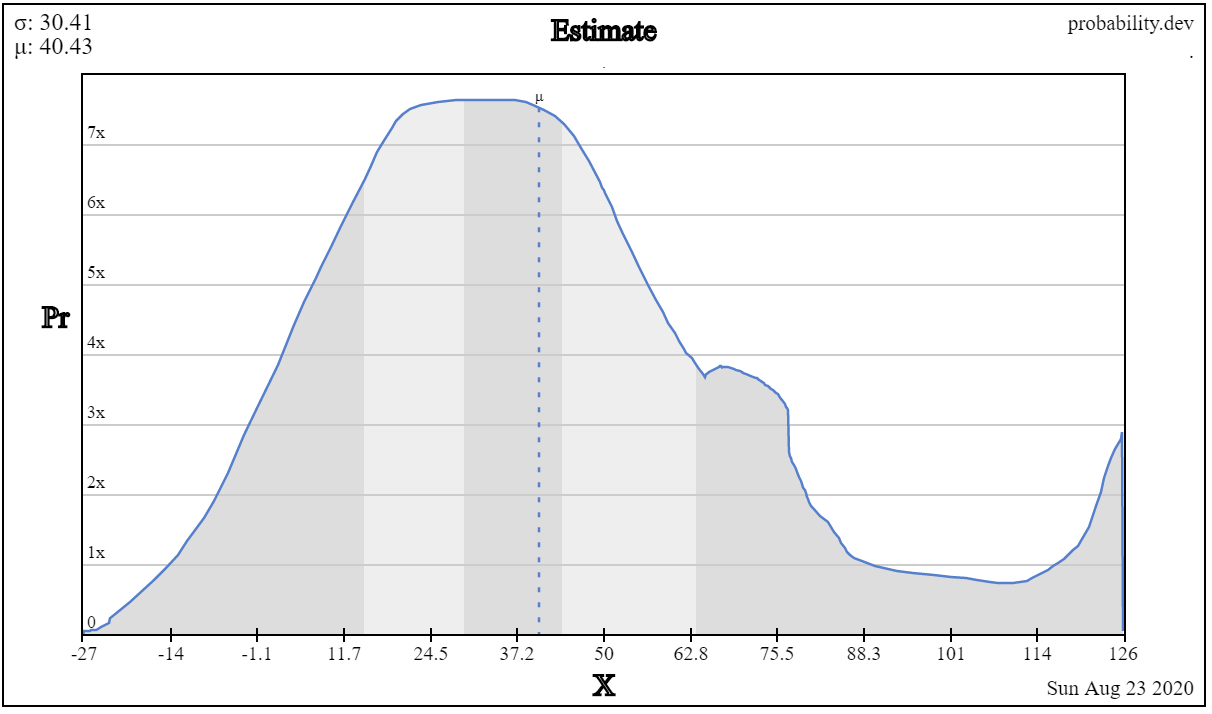probability.dev

## Ought’s elicit

Ought’s elicit lets you provide quantiles like my tool, or equivalently bins with some probability mass in each bin4. The resulting distribution is by default piecewise uniform (the cdf is piecewise linear), but it’s possible to apply smoothing. It has all the features I want, the drawback is that it only supports bounded distributions5.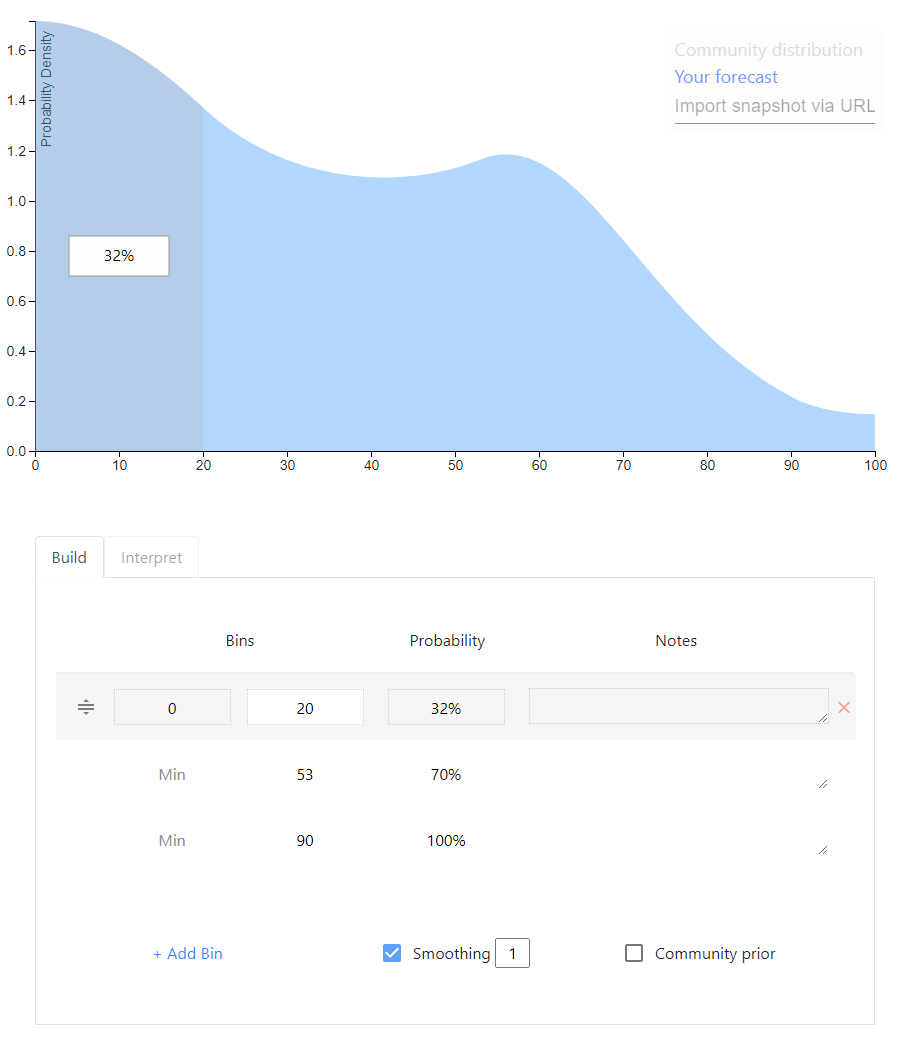Elicit

## Mixtures

A meta-level approach that can be applied to any of the above is to allow the user to specify a mixture distribution, a weighted average of distributions. For example, 1/3 weight on a normal(5,5) and 2/3 weight on a lognormal(1,0.75). My opinion on mixtures is that they are good if the user is thinking about the event disjunctively; for example, she may be envisioning two possible scenarios, each of which she has a distribution in mind for. But on Metaculus and Foretold my impression is that mixtures are often used to indirectly achieve a single distribution whose rough shape the user had in mind originally.

# The future

This is an exciting space with many recent developments. Guesstimate, Metaculus, Elicit and the metalog distribution have all been created in the last 5 years.

1. For the quantile function expression, see Keelin 2016, definition 1. The fact that this is in closed form means, first, that sampling randomly from the distribution is computationally trivial. We can use the inverse transform method: we take random samples from a uniform distribution over $$[0,1]$$ and plug them into the quantile function. Second, plotting the CDF for a certain range of probabilities (e.g. from 1% to 99%) is also easy.

The expression for the PDF is unusual in that it is a function of the cumulative probability $$p \in (0,1)$$, instead of a function of values of the random variable. See Keelin 2016, definition 2. As Keelin explains (p. 254), to plot the PDF as is customary we can use the quantile function $$q(p)$$ on the horizontal axis and the PDF expression $$f(p)$$ on the vertical axis, and vary $$p$$ in, for example, $$[0.01,0.99]$$ to produce the corresponding values on both axes.

Hence, for (i) querying the quantile function of the fitted metalog, sampling, and plotting the CDF, and (ii) plotting the PDF, everything can be done in closed form.

To query the CDF, however, numerical equation solving is applied. Since the quantile function is differentiable, Newton’s method can be applied and is fast. (Numerical equation solving is also used to query the PDF as a function of values of the random variable – but I don’t see why one would need densities except for plotting.)

2. In most cases, there exists a metalog whose CDF passes through all the provided quantiles exactly. In that case, there exists an expression of the metalog parameters that is in closed form as a function of the quantiles (“$$a = Y^{−1}x$$”, Keelin 2016, p. 253. Keelin denotes the metalog parameters $$a$$, the matrix $$Y$$ is a simple function of the quantiles’ y-coordinates, and the vector $$x$$ contains the quantiles’ x-coordinates. The metalog parameters $$a$$ are the numbers that are used to modify the logistic quantile function. This modification is done according to equation 6 on p. 254.)

If there is no metalog that fits the quantiles exactly (i.e. the expression for $$a$$ above does not imply a valid probability distribution), we have to use optimization to find the feasible metalog that fits the quantiles most closely. In this software implementation, “most closely” is defined as minimizing the absolute differences between the quantiles and the CDF (see here for more discussion).

In my experience, if a small number of quantiles describing a PDF with sharp peaks are provided, the closest feasible metalog fit to the quantiles may not pass through all the quantiles exactly.

3. Drawing the PDF instead of the CDF makes it difficult to hit quantiles. But drawing the CDF would probably be less intuitive – I often have the rough shape of the PDF in mind, but I never have intuitions about the rough shape of the CDF. The canvas-based approach also runs into difficulty with the tail of unbounded distributions. Overall I think it’s very cool but I haven’t found it that practical.

4. To provide quantiles, simply leave the Min field empty – it defaults to the left bound of the distribution.

5. I suspect this is a fundamental problem of the approach of starting with piecewise uniforms and adding smoothing. You need the tails of the CDF to asymptote towards 0 and 1, but it’s hard to find a mathematical function that does this while also (i) having the right probability mass under the tail (ii) stitching onto the piecewise uniforms in a natural way. I’d love to be proven wrong, though; the user interface and user experience on Elicit are really nice. (I’m aware that Elicit allows for ‘open-ended’ distributions, where probability mass can be assigned to an out-of-bounds outcome, but one cannot specify how that mass is distributed inside the out-of-bounds interval(s). So there is no true support for unbounded distributions. The ‘out-of-bounds’ feature exists because Elicit seems to be mainly intended as an add-on to Metaculus, which supports such ‘open-ended’ distributions but no truly unbounded ones.)

]]>
Debugging surprising behavior in SciPy numerical integration2020-07-01T00:00:00+00:002020-07-01T00:00:00+00:00https://bayes.net/bayes .highlight{ width: 125%; max-width: 95vw; min-width: 100%; position: absolute; left: 50%; transform: translateX(-50%); position: relative; }

I wrote a Python app to apply Bayes’ rule to continuous distributions. It looks like this:

Screenshot

I’m learning a lot about numerical analysis from this project. The basic idea is simple:

def unnormalized_posterior_pdf(x):
return prior.pdf(x)*likelihood.pdf(x)

# integrate unnormalized_posterior_pdf over the reals

def posterior_pdf(x):
return unnormalized_posterior_pdf(x)/normalization_constant


However, when testing my code on complicated distributions, I ran into some interesting puzzles.

A first set of problems was caused by the SciPy numerical integration routines that my program relies on. They were sometimes returning incorrect results or RuntimeErorrs. These problems appeared when the integration routines had to deal with ‘extreme’ values: small normalization constants or large inputs into the cdf function. I eventually learned to hold the integration algorithm’s hand a little bit and show it where to go.

A second set of challenges had to do with how long my program took to run: sometimes 30 seconds to return the percentiles of the posterior distribution. While 30 seconds might be acceptable for someone who desperately needed that bayesian update, I didn’t want my tool to feel like a punch card mainframe. I eventually managed to make the program more than 10 times faster. The tricks I used all followed the same strategy. In order to make it less expensive to repeatedly evaluate the posterior’s cdf by numerical integration, I tried to find ways to make the interval to integrate narrower.

You can follow along with all the tests described in this post using this file, whereas the code doing the calculations for the webapp is here.

# Small normalization constants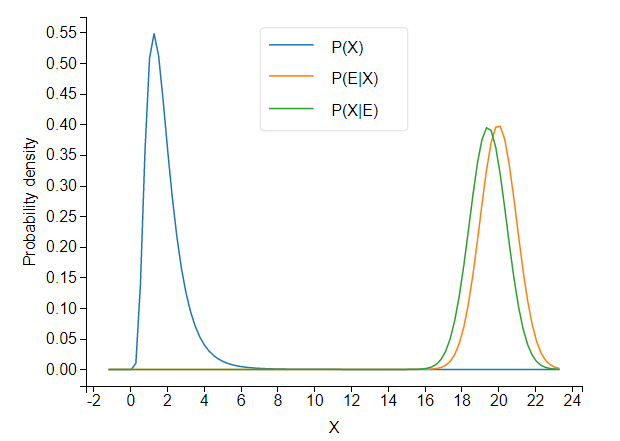When the prior and likelihood are far apart, the unnormalized posterior takes tiny values.

It turns out that SciPy’s integration routine, integrate.quad, (incidentally, written in actual Fortran!) has trouble integrating such a low-valued pdf.

prior = stats.lognorm(s=.5,scale=math.exp(.5)) # a lognormal(.5,.5) in SciPy notation
likelihood = stats.norm(20,1)

class Posterior_scipyrv(stats.rv_continuous):
def __init__(self,d1,d2):
super(Posterior_scipyrv, self).__init__()
self.d1= d1
self.d2= d2

def unnormalized_pdf(self,x):
return self.d1.pdf(x) * self.d2.pdf(x)

def _pdf(self,x):
return self.unnormalized_pdf(x)/self.normalization_constant

posterior = Posterior_scipyrv(prior,likelihood)

print('normalization constant:',posterior.normalization_constant)
print("CDF values:")
for i in range(30):
print(i,posterior.cdf(i))


The cdf converges to… 52,477. This is not so good.

Because the cdf does converge, but to an incorrect value, we can conclude that the normalization constant is to blame. Because the cdf converges to a number greater than 1, posterior.normalization_constant, about 3e-12, is an underestimate of the true value.

If we shift the likelihood distribution just a little bit to the left, to likelihood = stats.norm(18,1), the cdf converges correctly, and we get a normalization constant of about 6e-07. Obviously, the normalization constant should not jump five orders of magnitude from 6e-07 to 3e-12 as a result of this small shift.

The program is not integrating the unnormalized pdf correctly.

Difficulties with integration usually have to do with the shape of the function. If your integrand zig-zags up and down a lot, the algorithm may miss some of the peaks. But here, the shape of the posterior is almost the same whether we use stats.norm(18,1) or stats.norm(20,1)1. So the problem really seems to occur once we are far enough in the tails of the prior that the unnormalized posterior pdf takes values below a certain absolute (rather than relative) threshold. I don’t yet understand why. Perhaps some of the values are becoming too small to be represented with standard floating point numbers.

This seems rather bizarre, but here’s a piece of evidence that really demonstrates that low absolute values are what’s tripping up the integration routine that calculates the normalization constant. We just multiply the unnormalized pdf by 10000 (which will cancel out once we normalize).

def unnormalized_pdf(self,x):
return 10000*self.d1.pdf(x) * self.d2.pdf(x)


Now the cdf converges to 1 perfectly (??!).

# Large inputs into cdf

We take a prior and likelihood that are unproblematically close together:

prior = stats.lognorm(s=.5,scale=math.exp(.5))# a lognormal(.5,.5) in SciPy notation
likelihood = stats.norm(5,1)
posterior = Posterior_scipyrv(prior,likelihood)

for i in range(100):
print(i,posterior.cdf(i))


At first, the cdf goes to 1 as expected, but suddenly all hell breaks loose and the cdf decreases to some very tiny values:

22 1.0000000000031484
23 1.0000000000095246
24 1.0000000000031442
25 2.4520867144186445e-09
26 2.7186998869943613e-12
27 1.1495658559228458e-15


What’s going on? When asked to integrate the pdf from minus infinity up to some large value like 25, quad doesn’t know where to look for the probability mass. When the upper bound of the integral is in an area with still enough probability mass, like 23 or 24 in this example, quad finds its way to the mass. But if you ask it to find a peak very far away, it fails.

A piece of confirmatory evidence is that if we make the peak spikier and harder to find, by setting the likelihood’s standard deviation to 0.5 instead of 1, the cdf fails earlier:

22 1.000000000000232
23 2.9116983489798973e-12


We need to hold the integration algorithm’s hand and show it where on the real line the peak of the distribution is located. In SciPy’s quad, you can supply the points argument to point out places ‘where local difficulties of the integrand may occur’, but only when the integration interval is finite. The solution I came up with is to split the interval into two halves.

def split_integral(f,splitpoint,integrate_to):
a,b = -np.inf,np.inf
if integrate_to < splitpoint:
# just return the integral normally
else:
integral_left = integrate.quad(f, a, splitpoint)
integral_right = integrate.quad(f, splitpoint, integrate_to)
return integral_left + integral_right


This definitely won’t work for every difficult integral, but should help for many cases where most of the probability mass is not too far from the splitpoint.

For splitpoint, a simple choice is the average of the prior and likelihood’s expected values.

class Posterior_scipyrv(stats.rv_continuous):
def __init__(self,d1,d2):
self.splitpoint = (self.d1.expect()+self.d2.expect())/2


We can now override the built-in cdf method, and specify our own method that uses split_integral:

class Posterior_scipyrv(stats.rv_continuous):
def _cdf(self,x):
return split_integral(self.pdf,self.splitpoint,x)


Now things run correctly:

22 1.0000000000000198
23 1.0000000000000198
24 1.0000000000000198
25 1.00000000000002
26 1.0000000000000202
...
98 1.0000000000000198
99 1.0000000000000193


# Defining support of posterior

So far I’ve only talked about problems that cause the program to return the wrong answer. This section is about a problem that only causes inefficiency, at least when it isn’t combined with other problems.

If you don’t specify the support of a continuous random variable in SciPy, it defaults to the entire real line. This leads to inefficiency when querying quantiles of the distribution. If I want to know the 50th percentile of my distribution, I call ppf(0.5). As I described previously, ppf works by numerically solving the equation $$cdf(x)=0.5$$. The ppf method automatically passes the support of the distribution into the equation solver and tells it to only look for solutions inside the support. When a distribution’s support is a subset of the reals, searching over the entire reals is inefficient.

To remedy this, we can define the support of the posterior as the intersection of the prior and likelihood’s support. For this we need a small function that calculates the intersection of two intervals.

def intersect_intervals(two_tuples):
d1 , d2 = two_tuples

d1_left,d1_right = d1,d1
d2_left,d2_right = d2,d2

if d1_right < d2_left or d2_right < d2_left:
raise ValueError("the distributions have no overlap")

intersect_left,intersect_right = max(d1_left,d2_left),min(d1_right,d2_right)

return intersect_left,intersect_right


We can then call this function:

class Posterior_scipyrv(stats.rv_continuous):
def __init__(self,d1,d2):
super(Posterior_scipyrv, self).__init__()
a1, b1 = d1.support()
a2, b2 = d2.support()

# 'a' and 'b' are scipy's names for the bounds of the support
self.a , self.b = intersect_intervals([(a1,b1),(a2,b2)])


To test this, let’s use a beta distribution, which is defined on $$[0,1]$$:

prior = stats.beta(1,1)
likelihood = stats.norm(1,3)


We know that the posterior will also be defined on $$[0,1]$$. By defining the support of the posterior inside the the __init__ method of Posterior_scipyrv, we give SciPy access to this information.

We can time the resulting speedup in calculating posterior.ppf(0.99):

print("support:",posterior.support())
s = time.time()
print("result:",posterior.ppf(0.99))
e = time.time()
print(e-s,'seconds to evalute ppf')

support: (-inf, inf)
result: 0.9901821216897447
3.8804399967193604 seconds to evalute ppf

support: (0.0, 1.0)
result: 0.9901821216904315
0.40013647079467773 seconds to evalute ppf


We’re able to achieve an almost 10x speedup, with very meaningful impact on user experience. For less extreme quantiles, like posterior.ppf(0.5), I still get a 2x speedup.

The lack of properly defined support causes only inefficiency if we continue to use split_integral to calculate the cdf. But if we leave the cdf problem unaddressed, it can combine with the too-wide support to produce outright errors.

For example, suppose we use a beta distribution again for the prior, but we don’t use the split integral for the cdf, and nor do we define the support of the posterior as $$[0,1]$$ instead of $${\rm I\!R}$$.

prior = stats.beta(1,1)
likelihood = stats.norm(1,3)

class Posterior_scipyrv(stats.rv_continuous):
def __init__(self,d1,d2):
super(Posterior_scipyrv, self).__init__()
self.d1= d1
self.d2= d2

def unnormalized_pdf(self,x):
return self.d1.pdf(x) * self.d2.pdf(x)

def _pdf(self,x):
return self.unnormalized_pdf(x)/self.normalization_constant

posterior = Posterior_scipyrv(prior,likelihood)

print("cdf values:")
for i in range(20):
print(i/5,posterior.cdf(i/5))


The cdf fails quickly now:

3.2 0.9999999999850296
3.4 0.0
3.6 0.0


When the integration algorithm is looking over all of $$(-\infty,3.4]$$, it has no way of knowing that all the probability mass is in $$[0,1]$$. The posterior distribution has only one big bump in the middle, so it’s not surprising that the algorithm misses it.

If we now ask the equation solver in ppf to find quantiles, without telling it that all the solutions are in $$[0,1]$$, it will try to evaluate points like cdf(4), which return 0 – but ppf is assuming that the cdf is increasing. This leads to catastrophe. Running posterior.ppf(0.5) gives a RuntimeError: Failed to converge after 100 iterations. At first I wondered why beta distributions would always give me RuntimeErrors…

# Optimization: CDF memoization

When we call ppf, the equation solver calls cdf for the same distribution many times. This suggests we could optimize things further by storing known cdf values, and only doing the integration from the closest known value to the desired value. This will result in the same number of integration calls, but each will be over a smaller interval (except the first). This is a form of memoization.

We can also squeeze out some additional speedup by considering the cdf to be 1 forevermore once it reaches values close to 1.

class Posterior_scipyrv(stats.rv_continuous):
def _cdf(self,x):
# exploit considering the cdf to be 1
# forevermore once it reaches values close to 1
for x_lookup in self.cdf_lookup:
if x_lookup < x and np.around(self.cdf_lookup[x_lookup],5)==1.0:
return 1

# check lookup table for largest integral already computed below x
sortedkeys = sorted(self.cdf_lookup ,reverse=True)
for key in sortedkeys:
#find the greatest key less than x
if key<x:
self.cdf_lookup[float(x)] = ret
return ret

# Initial run
ret = split_integral(self.pdf,self.splitpoint,x)
self.cdf_lookup[float(x)] = ret
return ret


If we return to our earlier prior and likelihood

prior = stats.lognorm(s=.5,scale=math.exp(.5)) # a lognormal(.5,.5) in SciPy notation
likelihood = stats.norm(5,1)


and make calls to ppf([0.1, 0.9, 0.25, 0.75, 0.5]), the memoization gives us about a 5x speedup:

memoization False
[2.63571613 5.18538207 3.21825988 4.56703016 3.88645864]
length of lookup table: 0
2.1609253883361816 seconds to evalute ppf

memoization True
[2.63571613 5.18538207 3.21825988 4.56703016 3.88645864]
length of lookup table: 50
0.4501194953918457 seconds to evalute ppf


These speed gains again occur over a range that makes quite a difference to user experience: going from multiple seconds to a fraction of a second.

# Optimization: ppf with bounds

In my webapp, I give the user some standard percentiles: 0.1, 0.25, 0.5, 0.75, 0.9.

Given that ppf works by numerical equation solving on the cdf, if we give the solver a smaller domain in which to look for the solutions, it should find them more quickly. When we calculate multiple percentiles, each percentile we calculate helps us close in on the others. If the 0.1 percentile is 12, we have a lower bound of 12 for on any percentile $$p>0.1$$. If we have already calculated a percentile on each side, we have both a lower and upper bound.

We can’t directly pass the bounds to ppf, so we have to wrap the method, which is found here in the source code. (To help us focus, I give a simplified presentation below that cuts out some code designed to deal with unbounded supports. The code below will not run correctly).

class Posterior_scipyrv(stats.rv_continuous):
def ppf_with_bounds(self, q, leftbound, rightbound):
left, right = self._get_support()

# SciPy ppf code to deal with case where left or right are infinite.
# Omitted for simplicity.

if leftbound is not None:
left = leftbound
if rightbound is not None:
right = rightbound

# brentq is the equation solver (from Brent 1973)
# _ppf_to_solve is simply cdf(x)-q, since brentq
# finds points where a function equals 0
return optimize.brentq(self._ppf_to_solve,left, right, args=q)


To get some bounds, we run the extreme percentiles first, narrowing in on the middle percentiles from both sides. For example in 0.1, 0.25, 0.5, 0.75, 0.9, we want to evaluate them in this order: 0.1, 0.9, 0.25, 0.75, 0.5. We store each of the answers in result.

class Posterior_scipyrv(stats.rv_continuous):
def compute_percentiles(self, percentiles_list):
result = {}
percentiles_list.sort()

# put percentiles in the order they should be computed
percentiles_reordered = sum(zip(percentiles_list,reversed(percentiles_list)), ())[:len(percentiles_list)] # see https://stackoverflow.com/a/17436999/8010877

def get_bounds(dict, p):
# get bounds (if any) from already computed results
keys = list(dict.keys())
keys.append(p)
keys.sort()
i = keys.index(p)
if i != 0:
leftbound = dict[keys[i - 1]]
else:
leftbound = None
if i != len(keys) - 1:
rightbound = dict[keys[i + 1]]
else:
rightbound = None
return leftbound, rightbound

for p in percentiles_reordered:
leftbound , rightbound = get_bounds(result,p)
res = self.ppf_with_bounds(p,leftbound,rightbound)
result[p] = np.around(res,2)

sorted_result = {key:value for key,value in sorted(result.items())}
return sorted_result


The speedup is relatively minor when calculating just 5 percentiles.

Using ppf bounds? True
total time to compute percentiles: 3.1997928619384766 seconds

Using ppf bounds? False
total time to compute percentiles: 3.306936264038086 seconds


It grows a little bit with the number of percentiles, but calculating a large number of percentiles would just lead to information overload for the user.

This was surprising to me. Using the bounds dramatically cuts the width of the interval for equation solving, but leads to only a minor speedup. Using fulloutput=True in optimize.brentq, we can see the number of function evaluations that brentq uses. This lets us see that the number of evaluations needed by brentq is highly non-linear in the width of the interval. The solver gets quite close to the solution very quickly, so giving it a narrow interval hardly helps.

Using ppf bounds? True
brentq looked between 0.0 10.0 and took 11 iterations
brentq looked between 0.52 10.0 and took 13 iterations
brentq looked between 0.52 2.24 and took 8 iterations
brentq looked between 0.81 2.24 and took 9 iterations
brentq looked between 0.81 1.73 and took 7 iterations
total time to compute percentiles: 3.1997928619384766 seconds

Using ppf bounds? False
brentq looked between 0.0 10.0 and took 11 iterations
brentq looked between 0.0 10.0 and took 10 iterations
brentq looked between 0.0 10.0 and took 10 iterations
brentq looked between 0.0 10.0 and took 10 iterations
brentq looked between 0.0 10.0 and took 9 iterations
total time to compute percentiles: 3.306936264038086 seconds


Brent’s method is a very efficient equation solver.

1. It has a very similar shape to the likelihood (because the likelihood has much lower variance than the prior).

]]>